# Plane Shapes

Have you noticed the shape of a pizza? It is round in shape.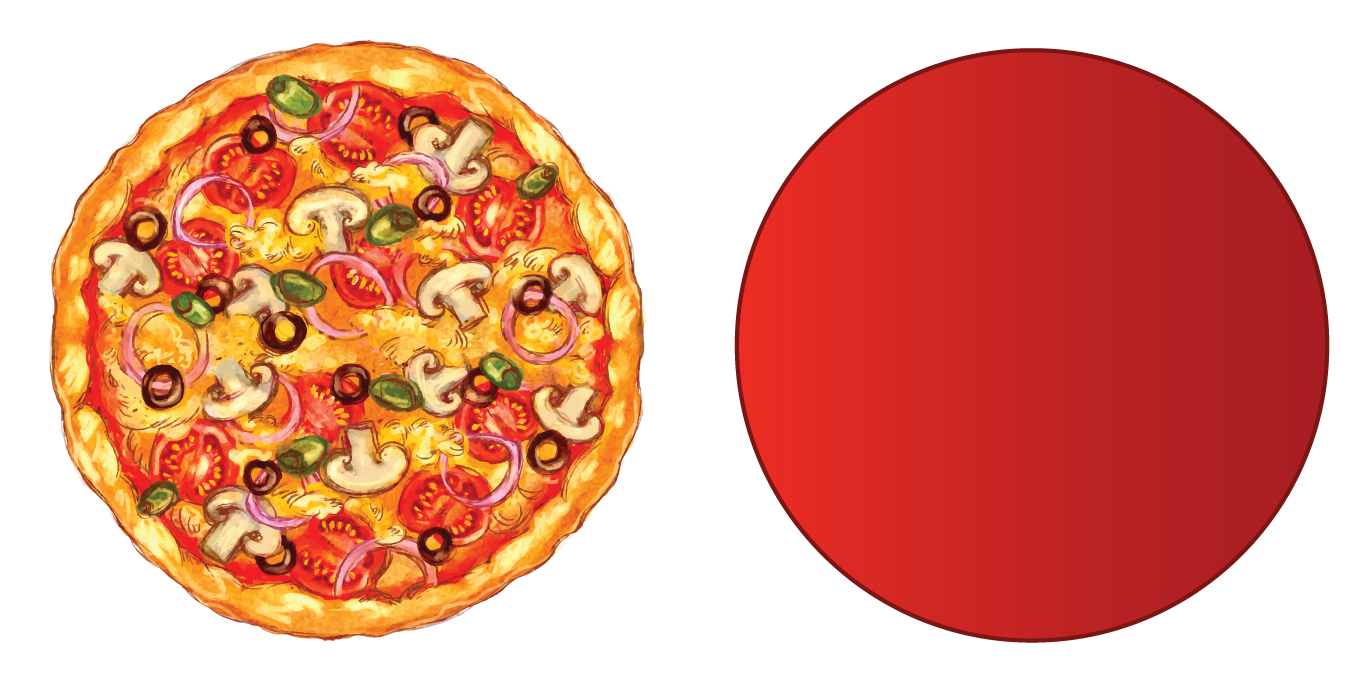If we cut out a slice from the pizza, the slice gets a triangular shape.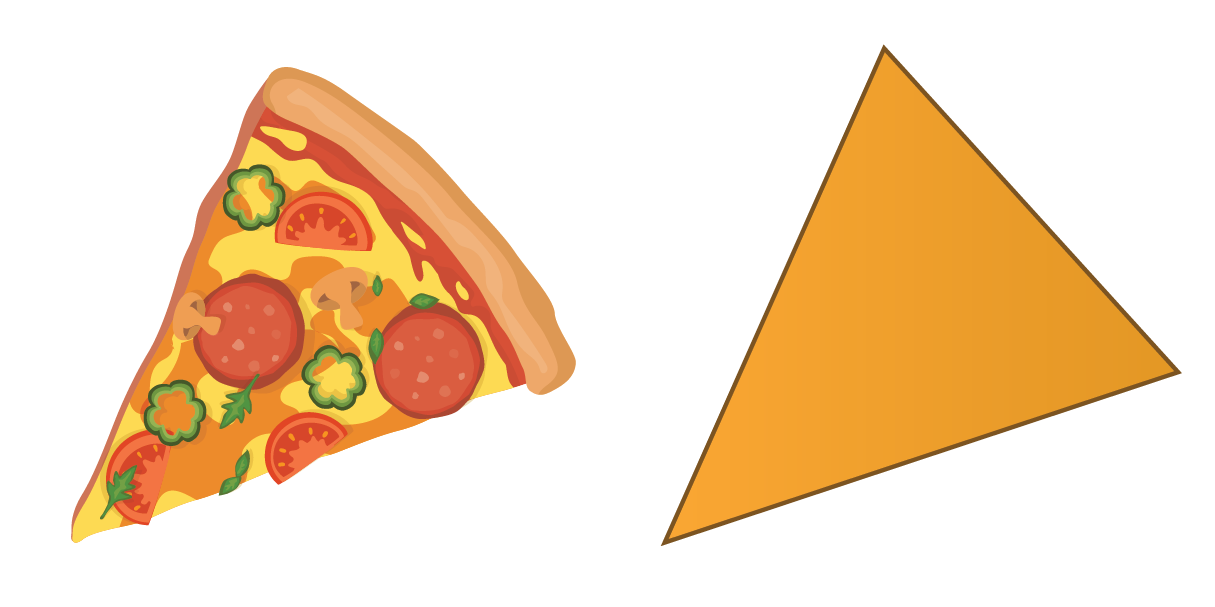In this lesson, you will learn all about plane shapes, types of plane shapes, and geometric shapes. You can check out the interactive simulations to know more about the lesson and try your hand at solving a few interesting practice questions at the end of the page. So, what are you waiting for?

Let's begin!

## Lesson Plan

 1 What Are Plane Shapes? 2 Important Notes 3 Solved Examples on Plane Shapes 4 Interactive Questions on Plane Shapes 5 Challenging Questions on Plane Shapes

## What Are Plane Shapes?

A plane shape is a two-dimensional closed figure that has no thickness.

A plane in geometry is a flat surface that extends into infinity in all directions.

A plane has no thickness. It is also known as a two-dimensional surface.

It has infinite width and length, zero thickness, and zero curvature.

It is actually difficult to imagine a plane in real life, there is nothing that we can use as a real example of a geometric plane.

We can see an example of a plane in coordinate geometry where the position of any given point on a plane is defined with the help of an ordered pair of numbers, or coordinates. Coordinates show the correct location of points in the plane.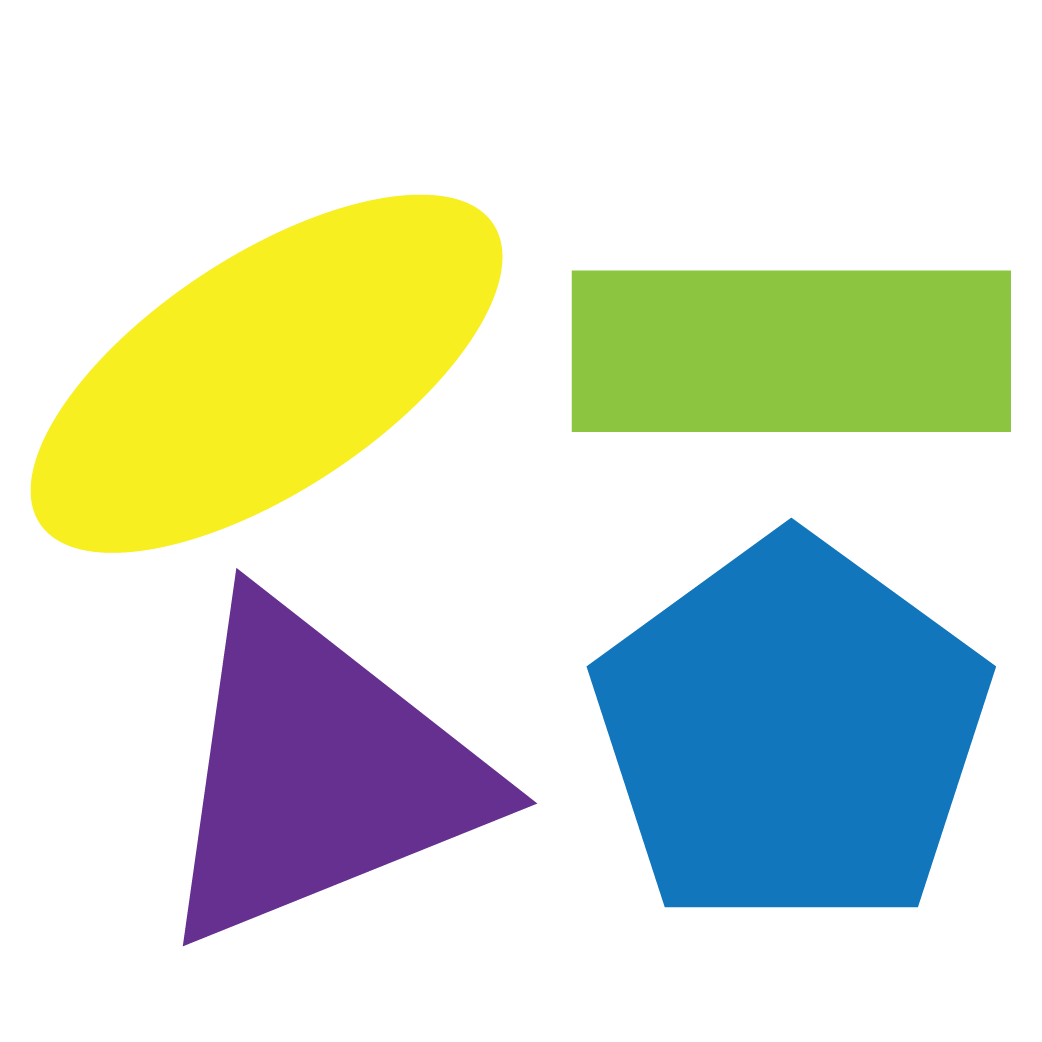## What Are Open and Closed Shapes?

A closed figure can be made of straight or curved lines. It has no opening. Given below is an example of a closed figure.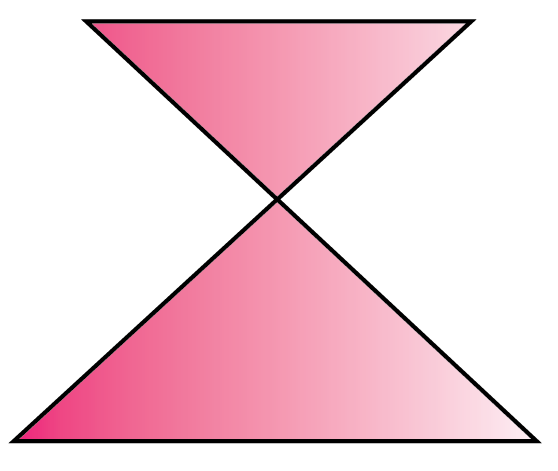An open figure is made of either straight or curved lines. However, it has at least one opening. Given below is an example of an open figure.## Sides and Corners

We have learned what plane shapes are and what they look like. Plane shapes are two dimensional, e.g., squares, triangles, and circles. The straight lines that make up a plane shape are called sides. The points where two sides meet are called corners.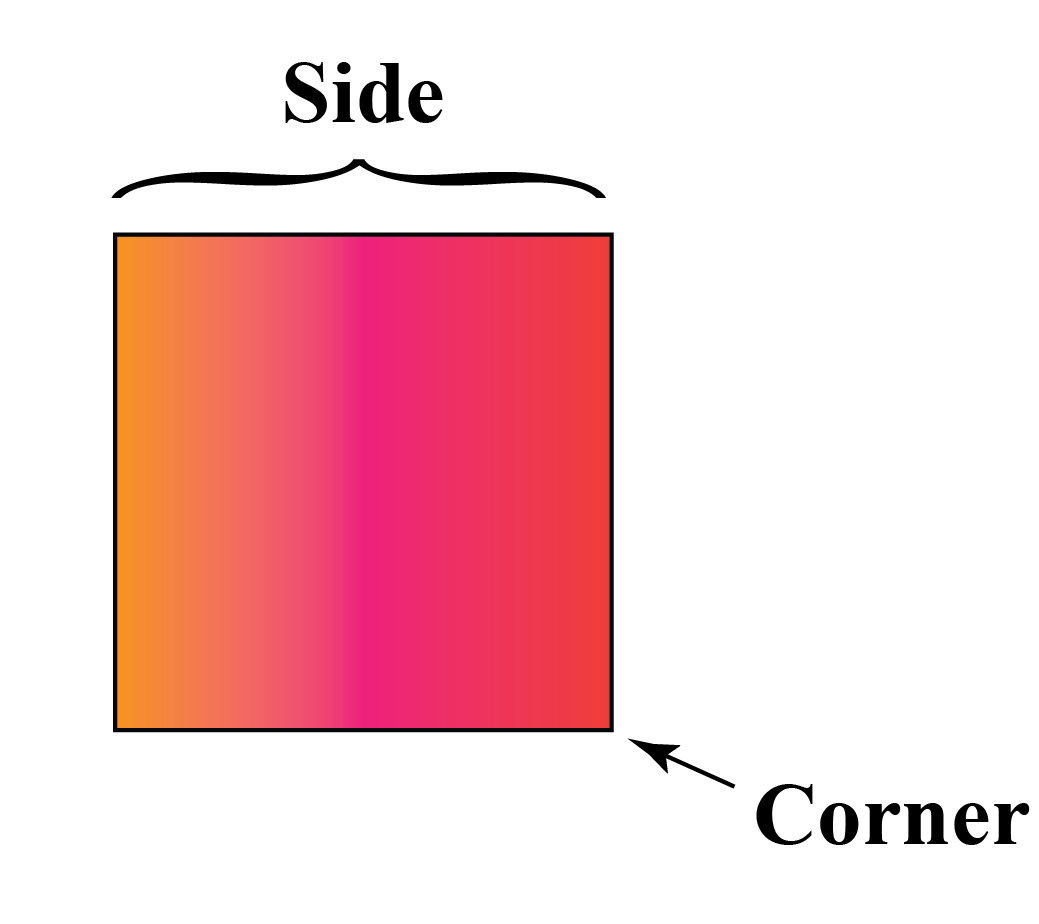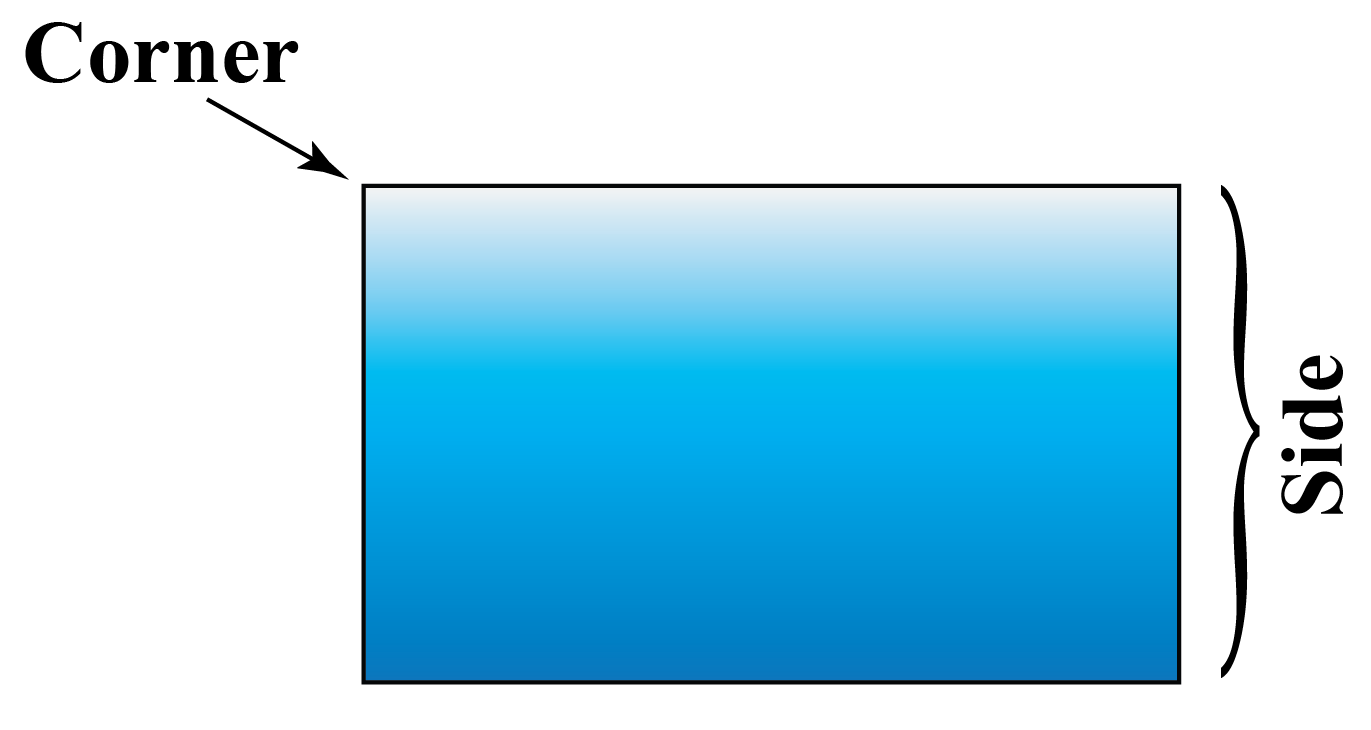Tips and Tricks
• Count the number of sides to identify the shapes
• Use paper cut-outs to visualize the shapes
• Use everyday objects trace the surface and identify the plane shapes

## What Are the Different 2D Shapes?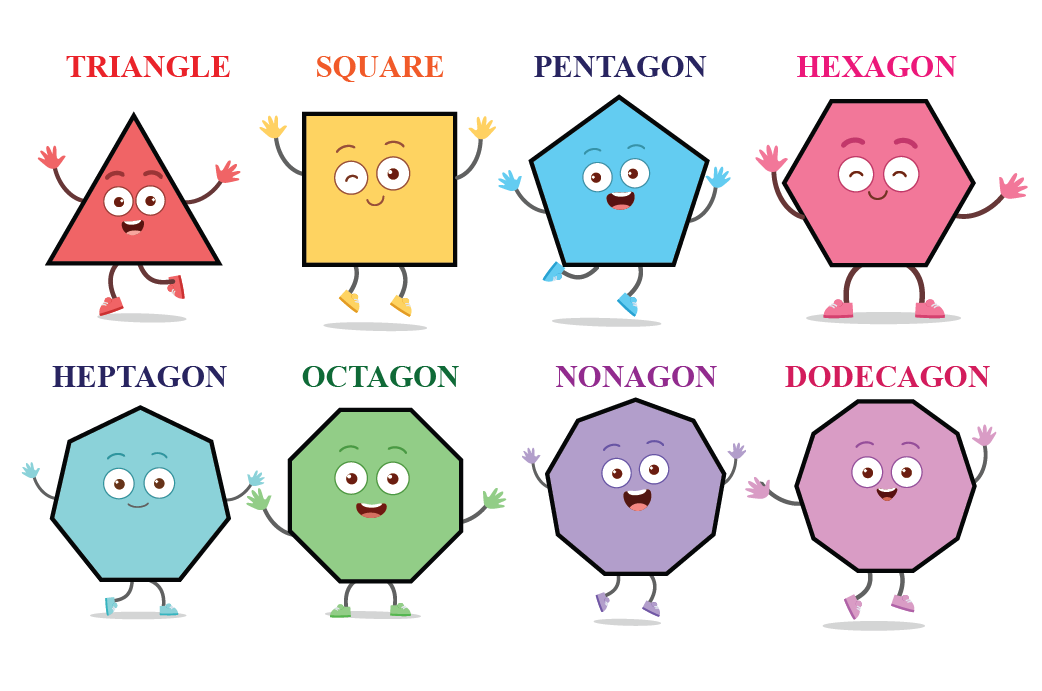Observe this sheet of paper.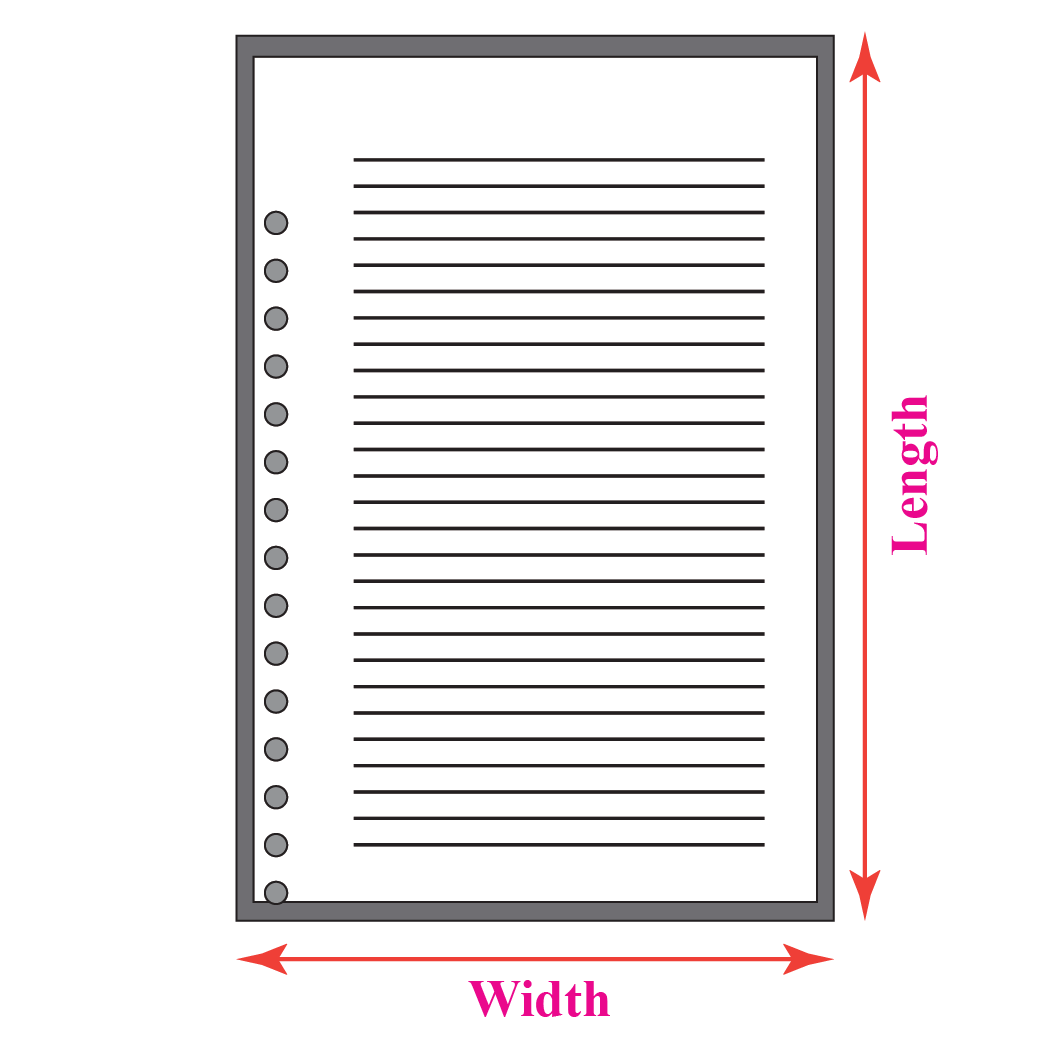It consists of a length and a width.

A two-dimensional shape does not have any depth.

2D shapes are closed shapes that are created by joining straight/curved lines together.

Explore different polygons below changing with the number of sides.

The common 2D shapes are:

 $$2\text{D}$$ Shapes Name Number of sides Image Triangle 3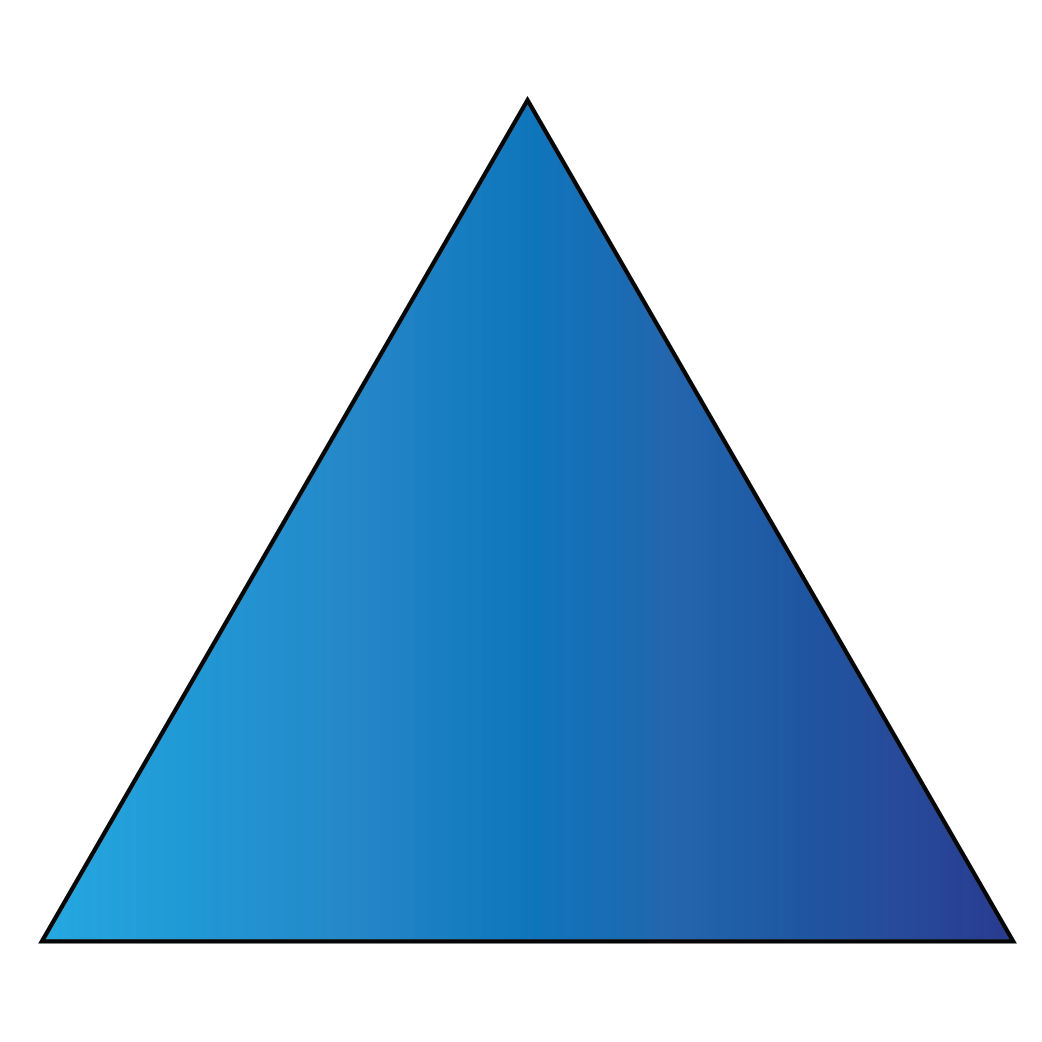Square 4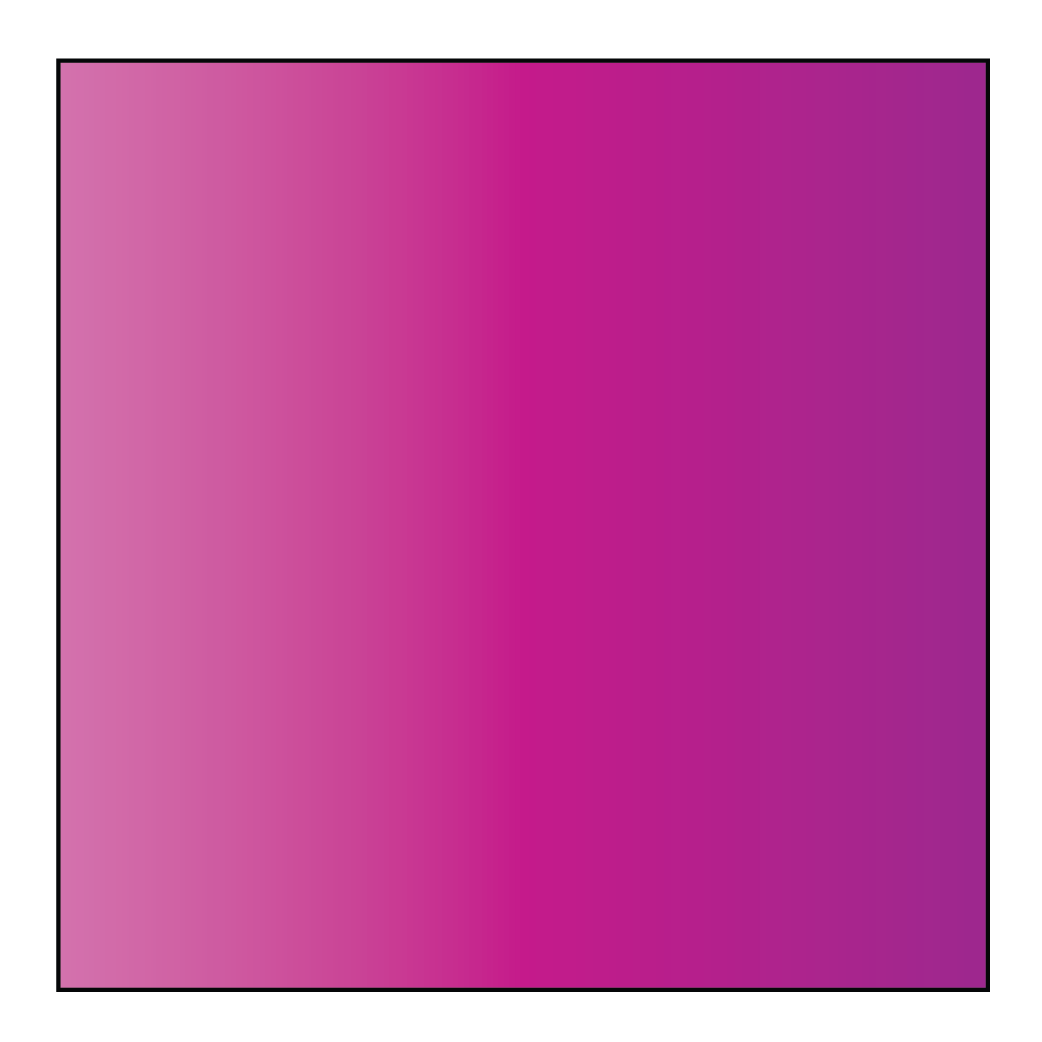Rhombus 4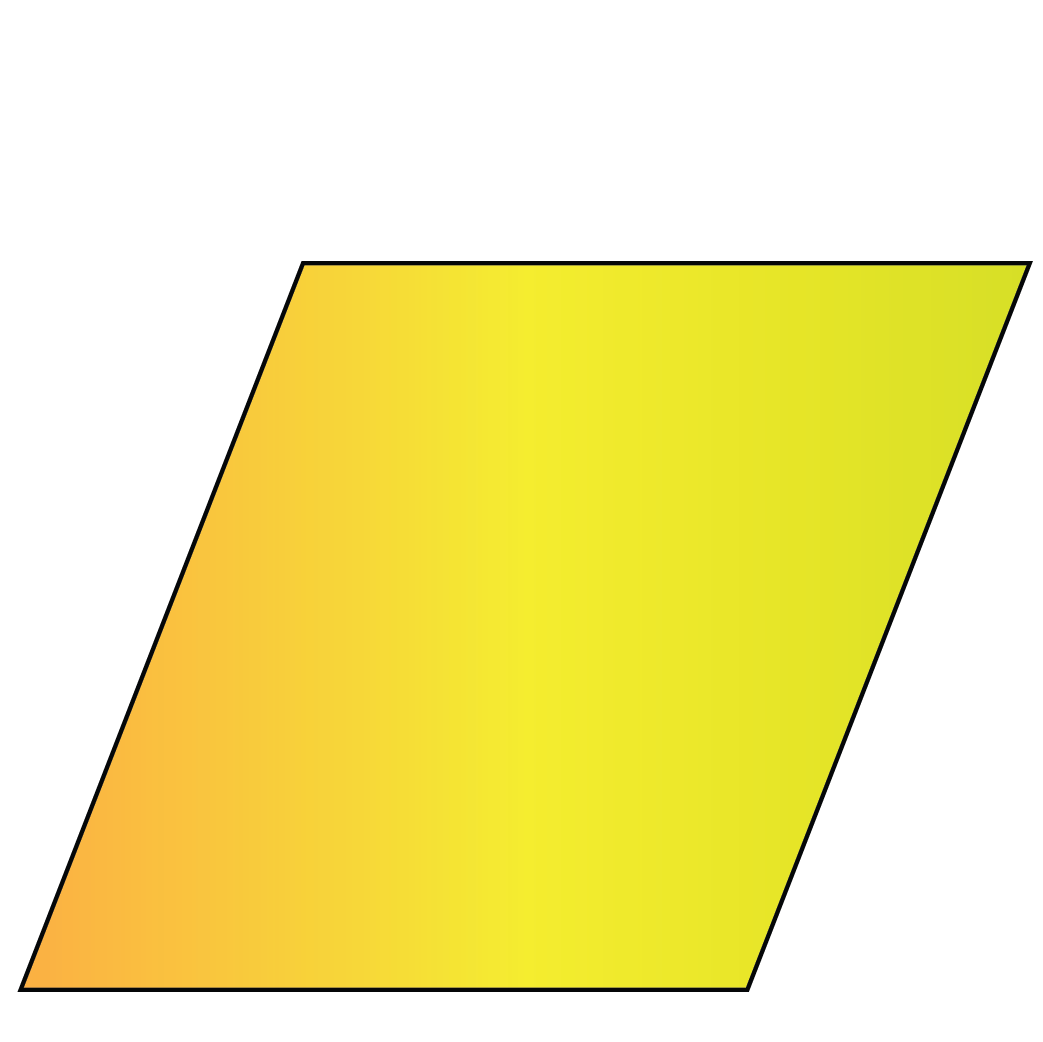Kite 4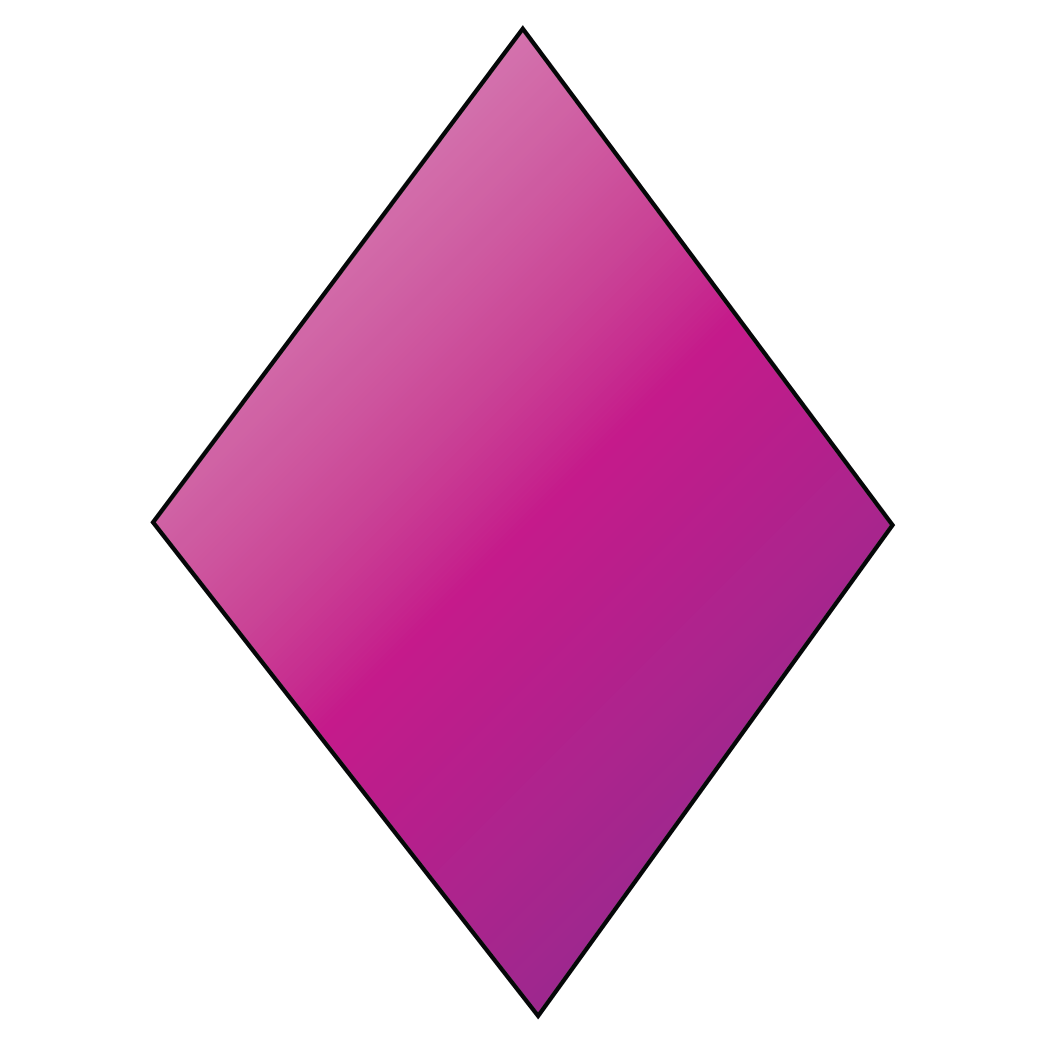Rectangle 4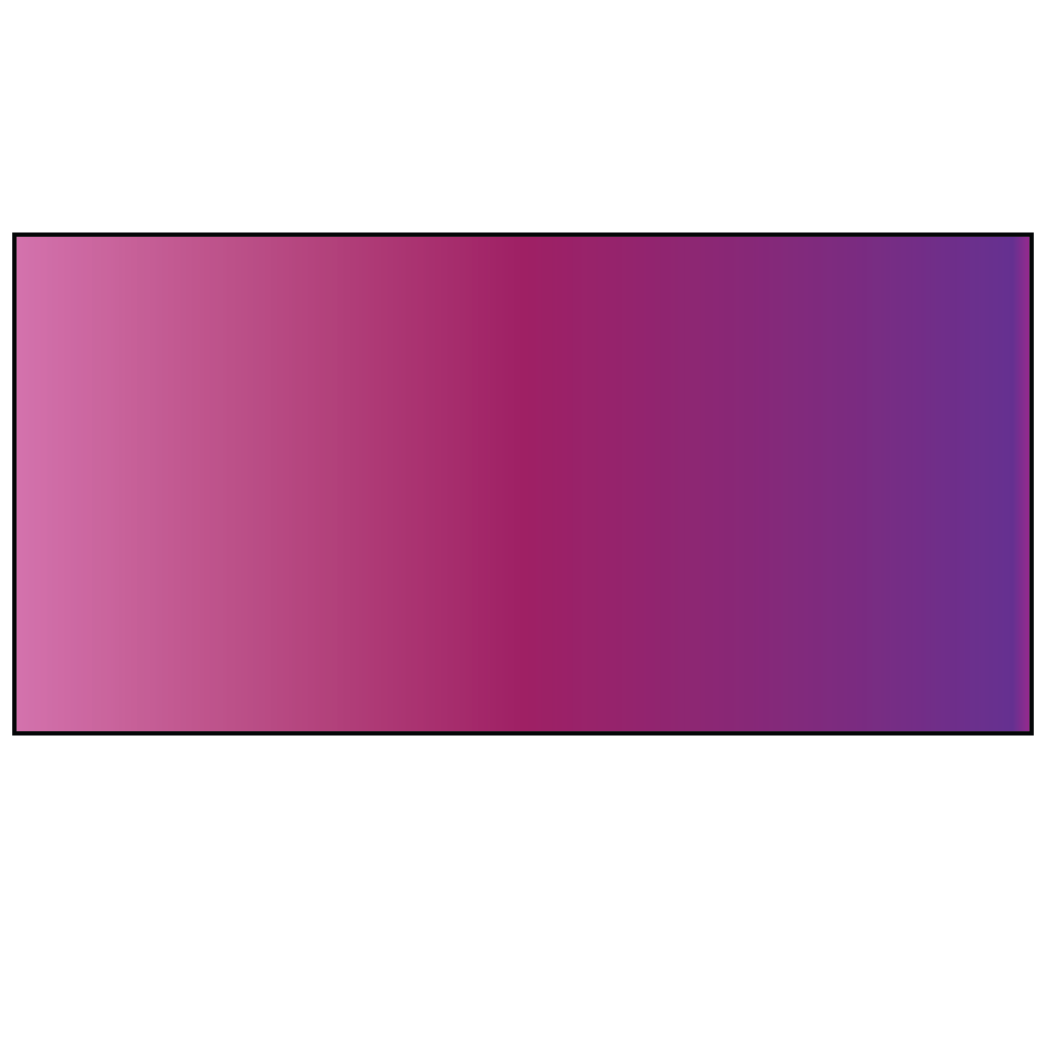Trapezium 4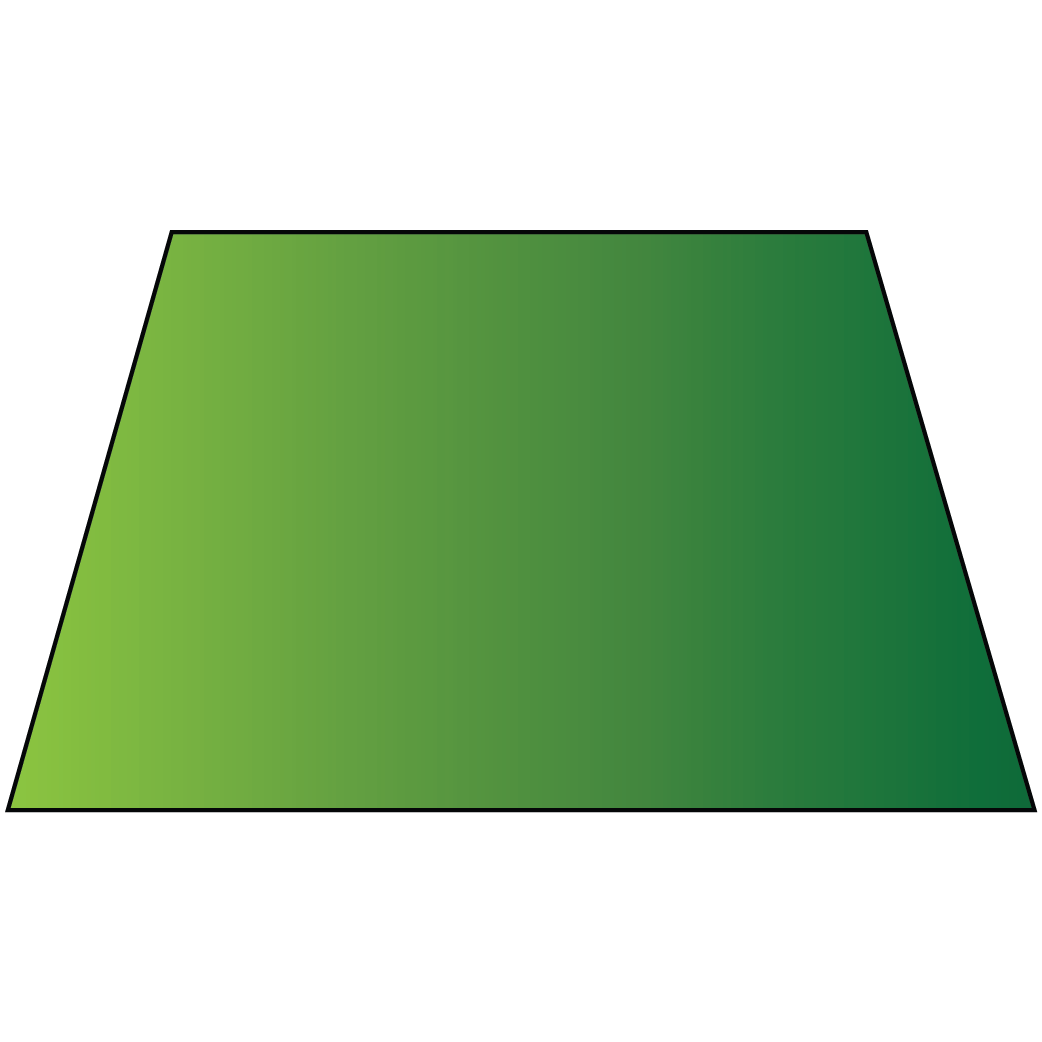Pentagon 5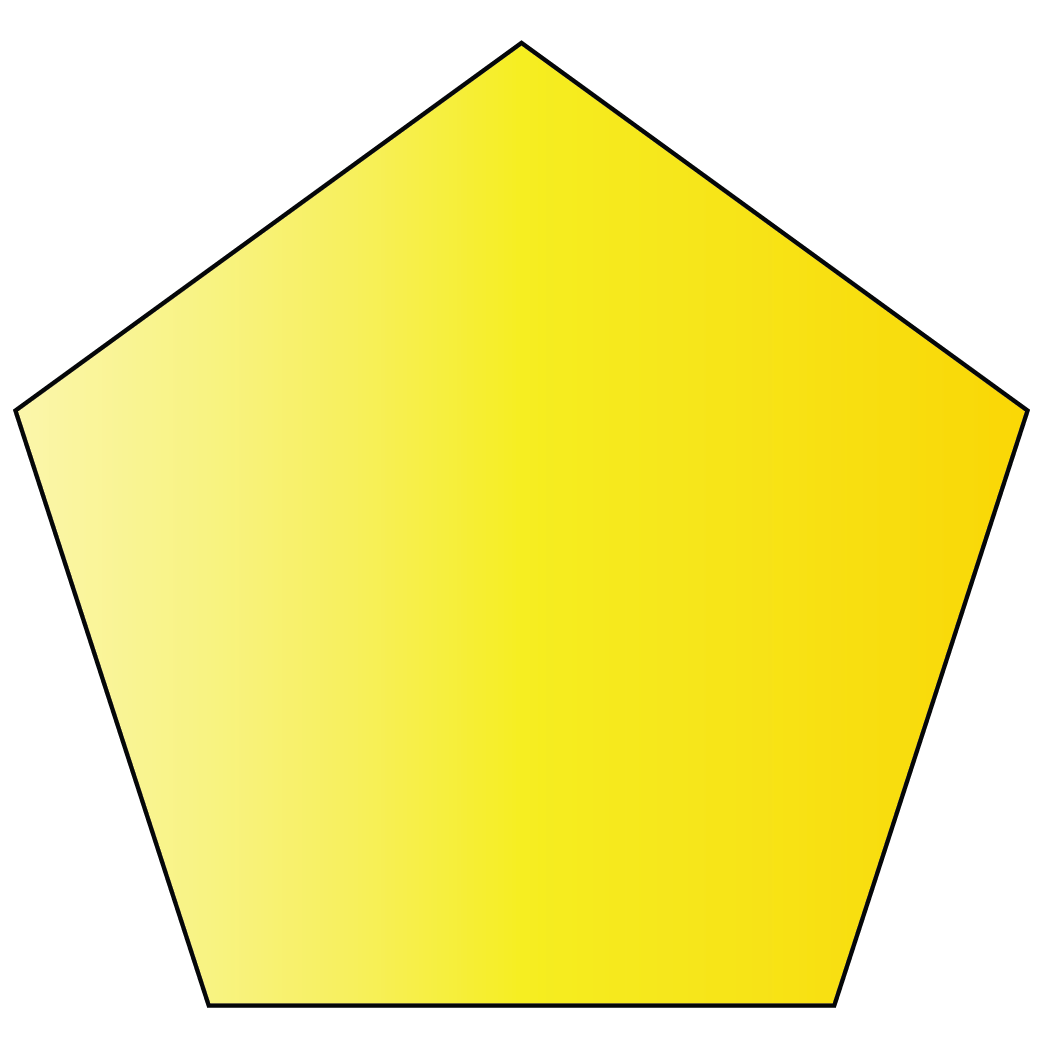Hexagon 6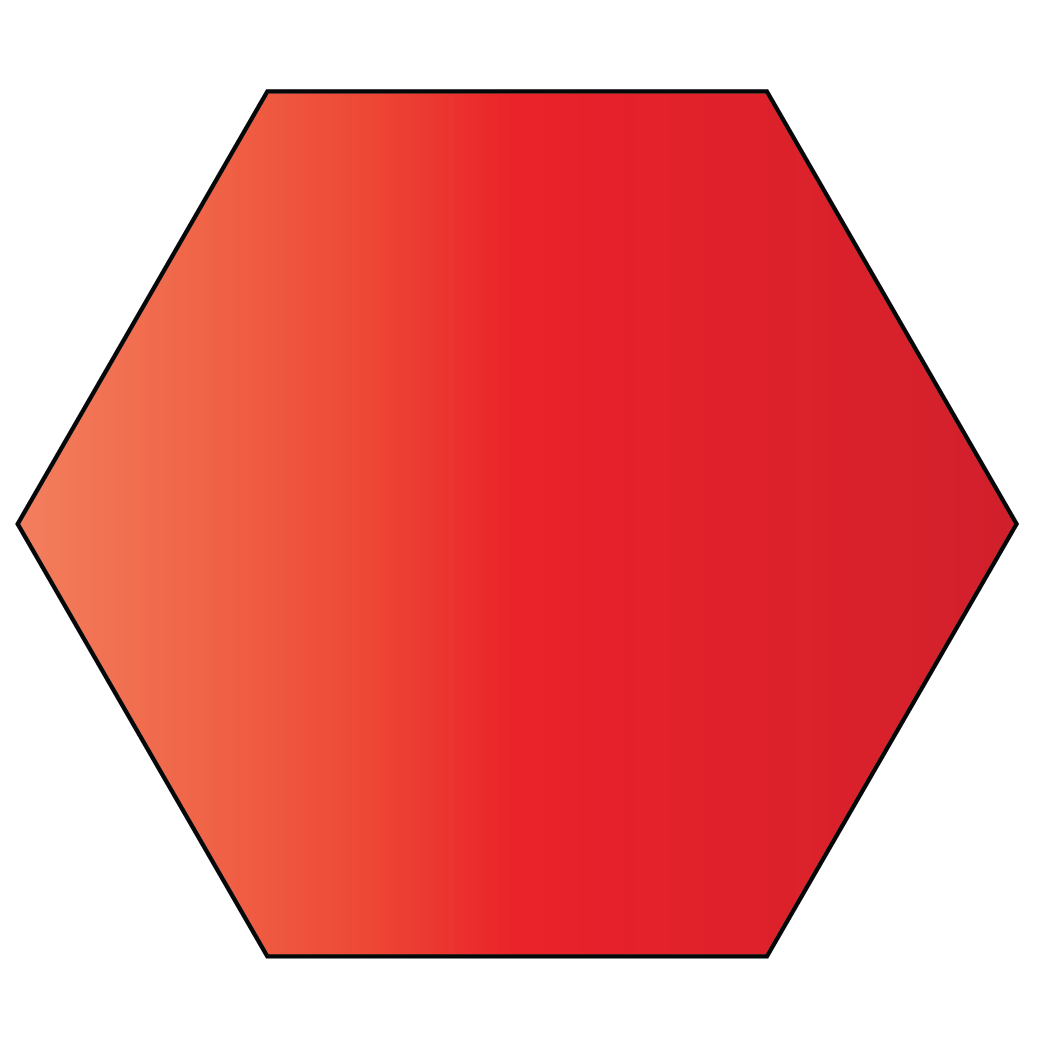Heptagon 7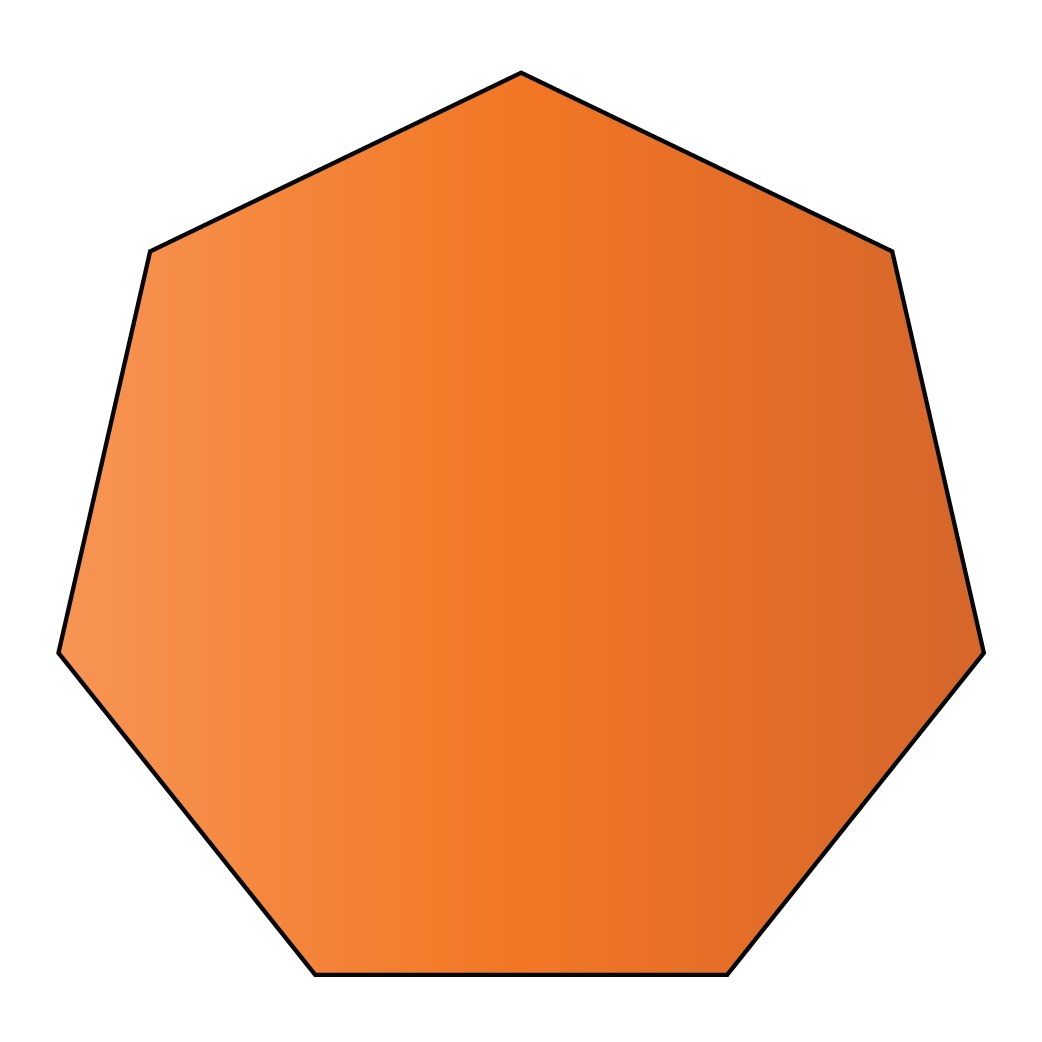Octagon 8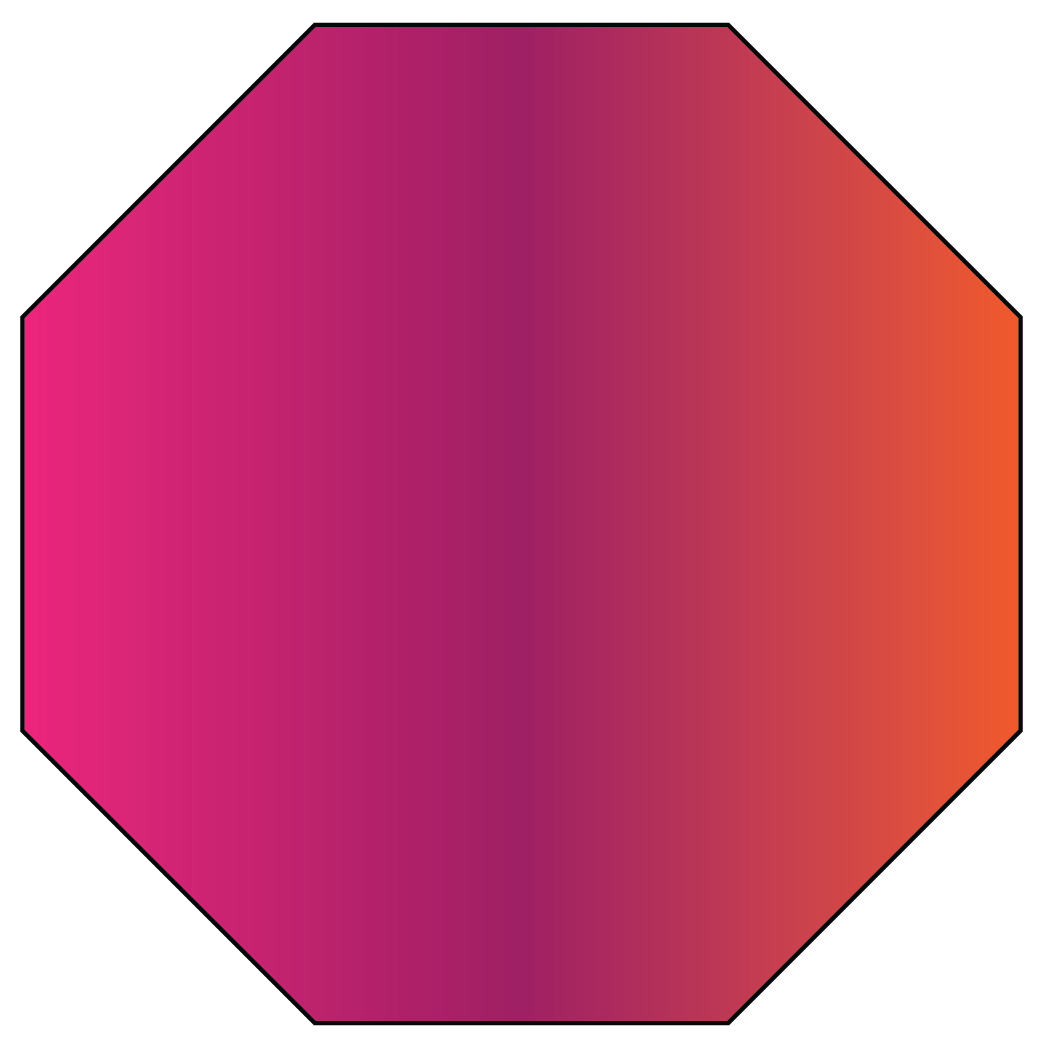Decagon 10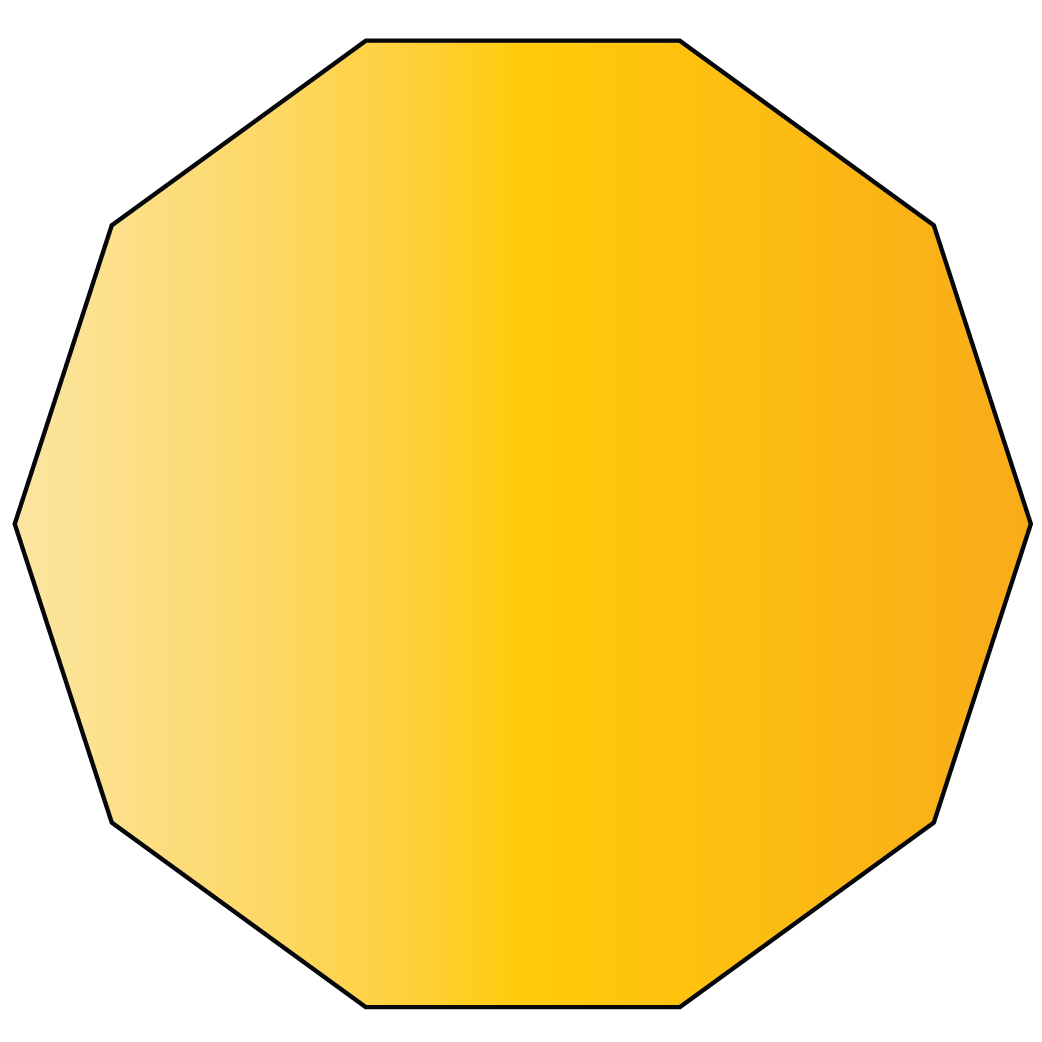Dodecagon 12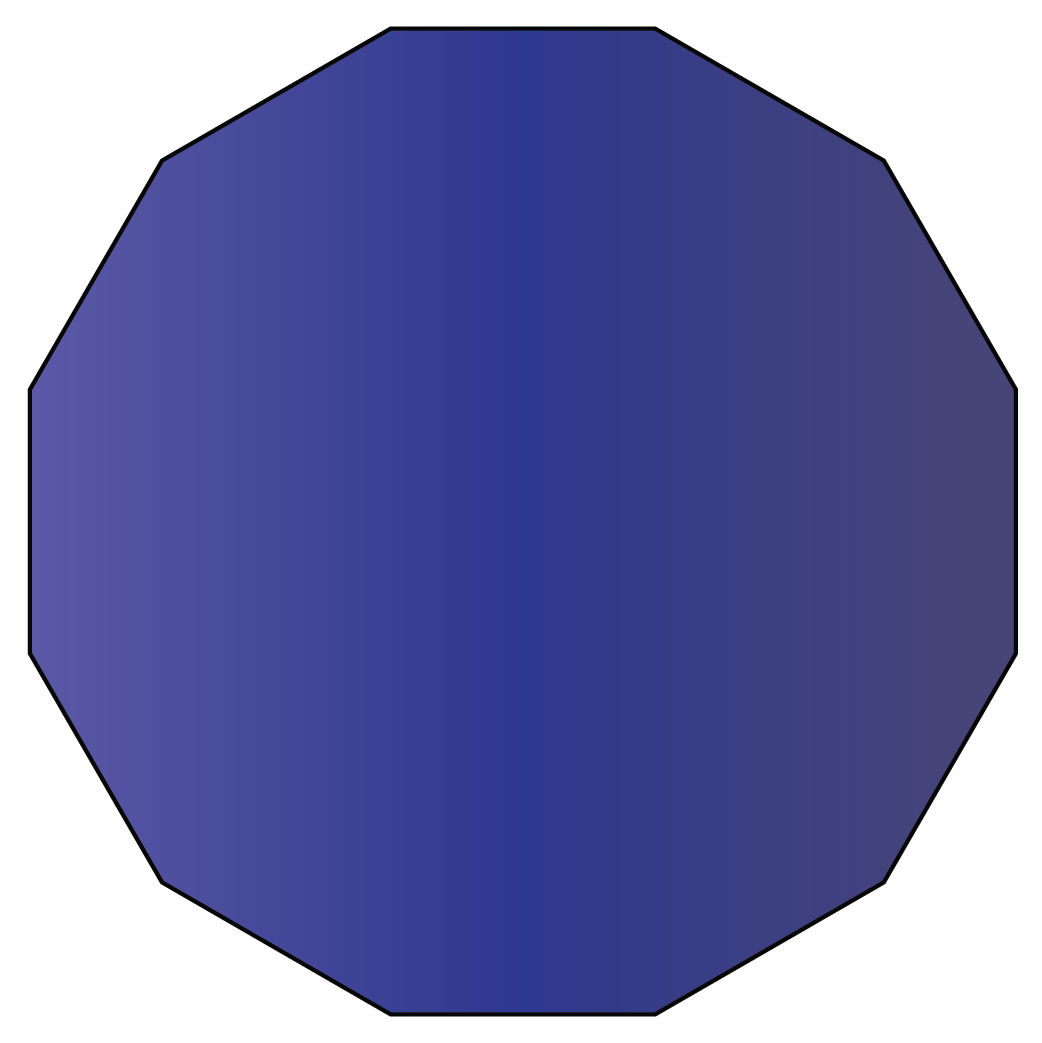Hexadecagon 16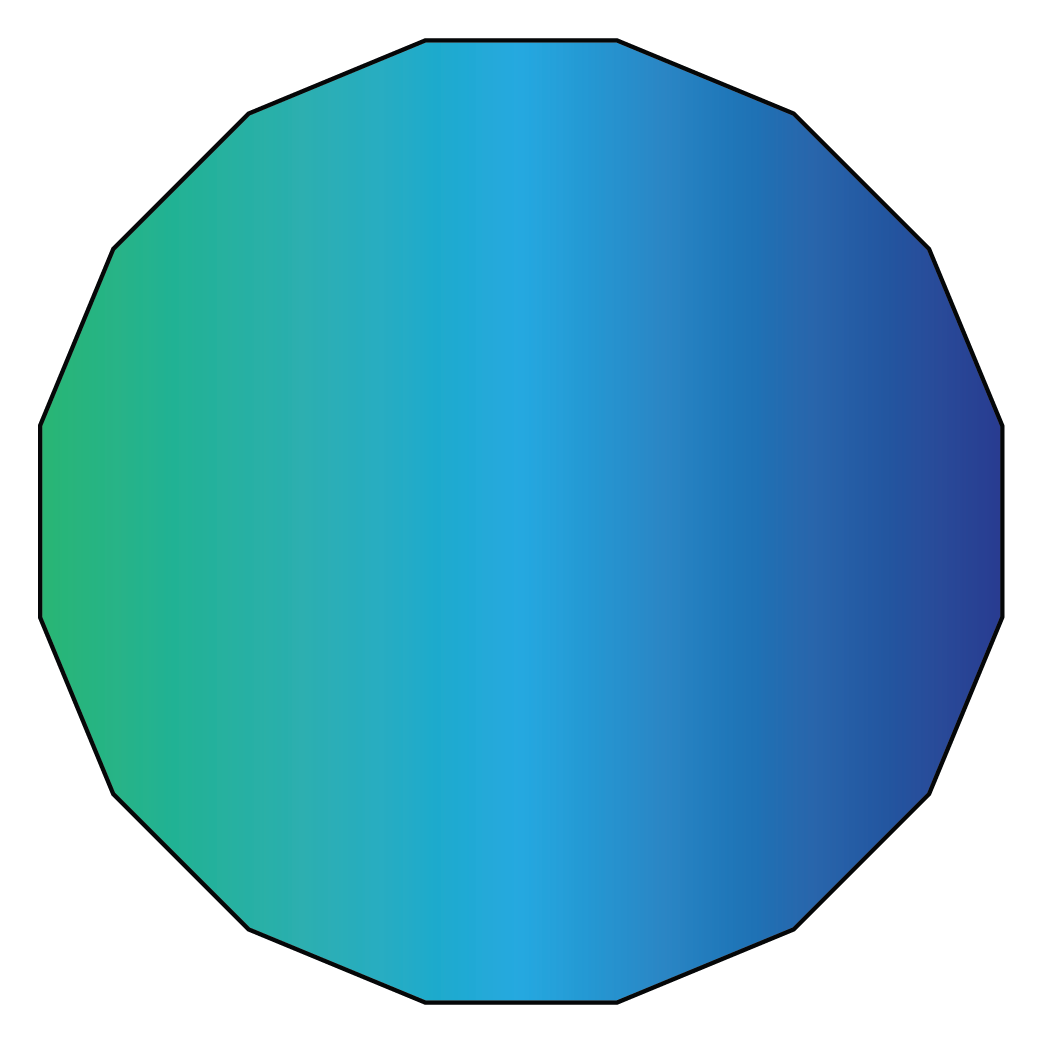Circle 0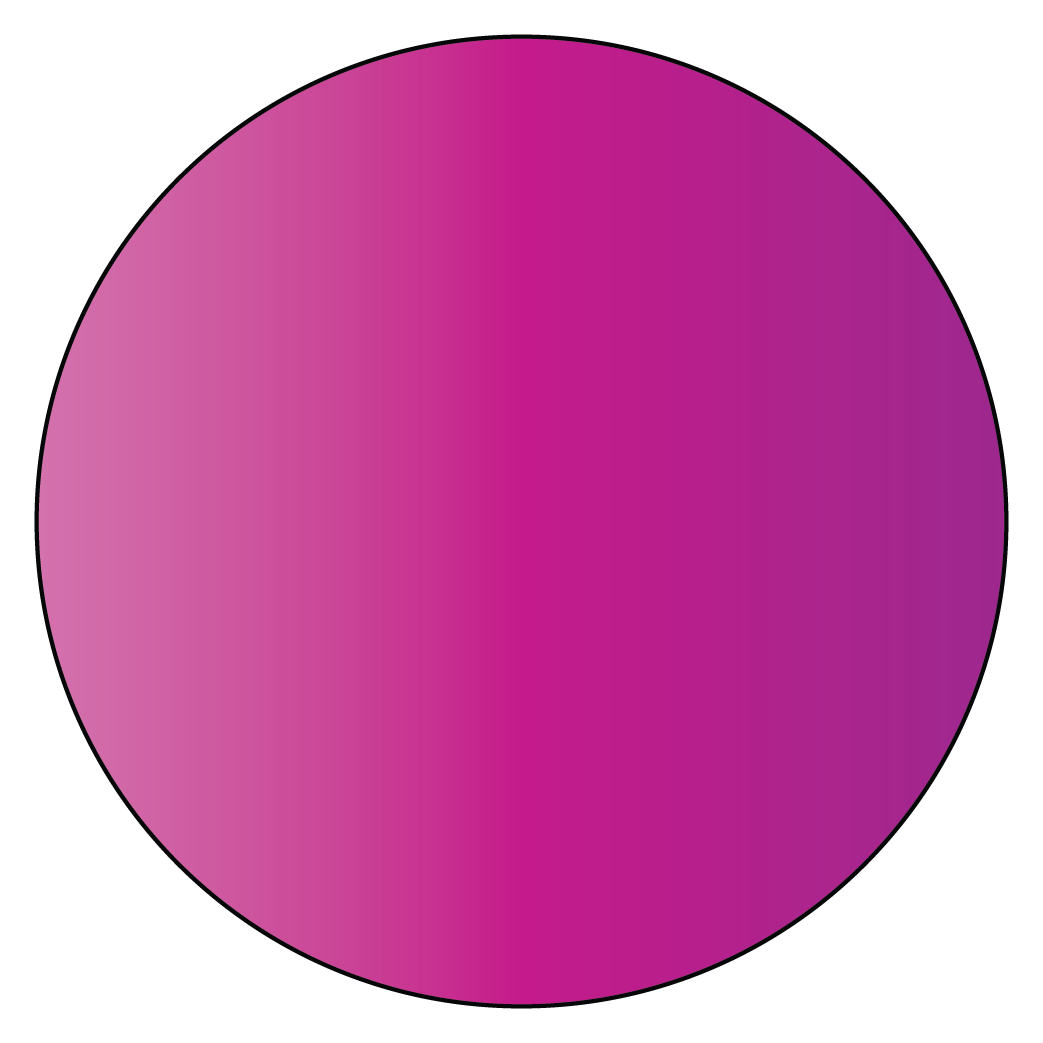## What Are Polygons?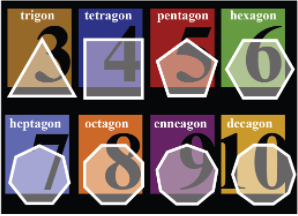In geometry, a polygon is a closed two-dimensional figure with three or more straight lines.

The Greek word ‘Polygon’ consists of Poly meaning ‘many’ and gon meaning ‘angle.’

We see many different polygons around us.

For example, Have you ever seen a honeycomb? Can you guess ideally, how many sides a honeycomb could have?

A honeycomb is hexagonal in shape. You can very well relate the shape of a 6 sided polygon with a honeycomb.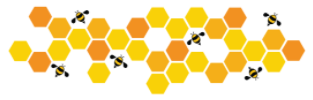The types of polygons are categorized into three major forms:

Let us look at a few different types of polygons with their sides:

Sides

Shape and Name of A Polygon

3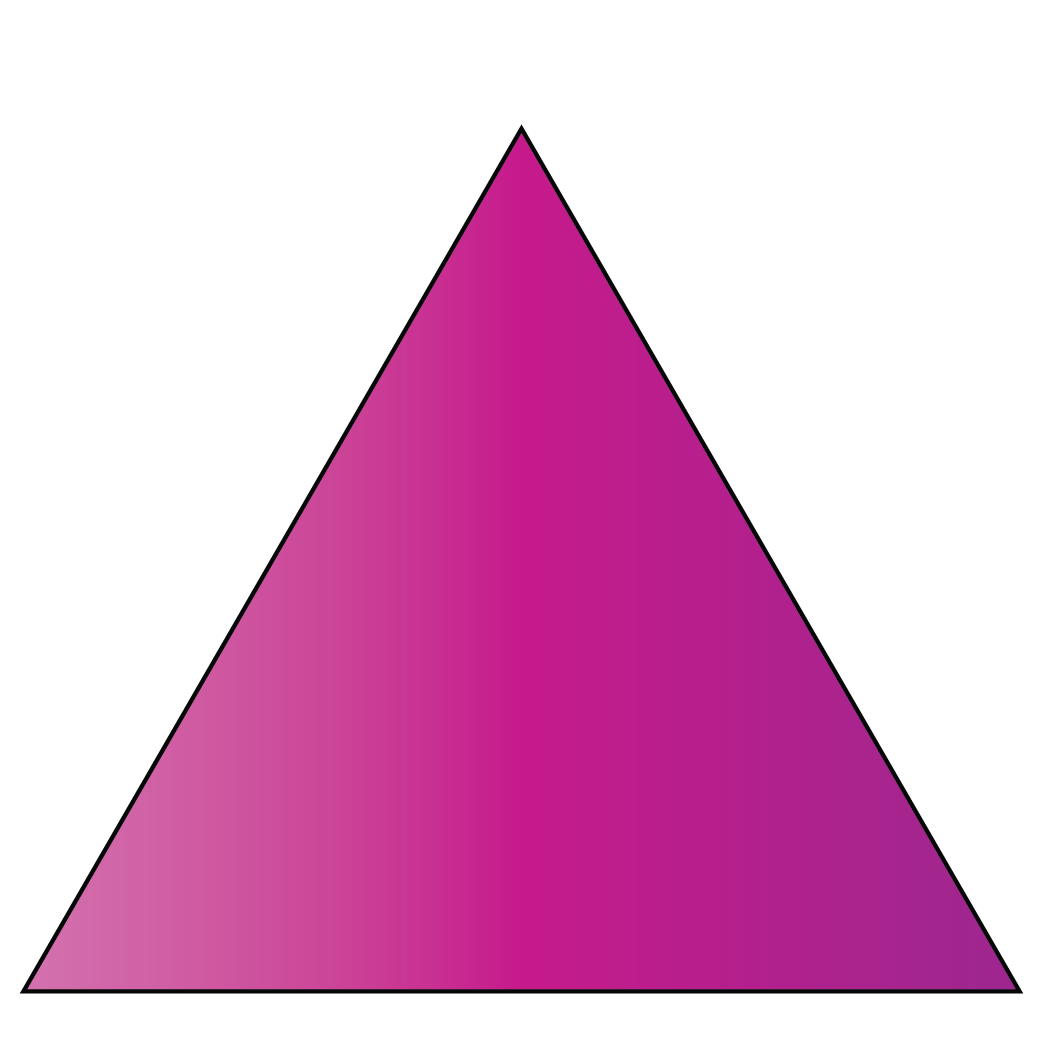Trigon

4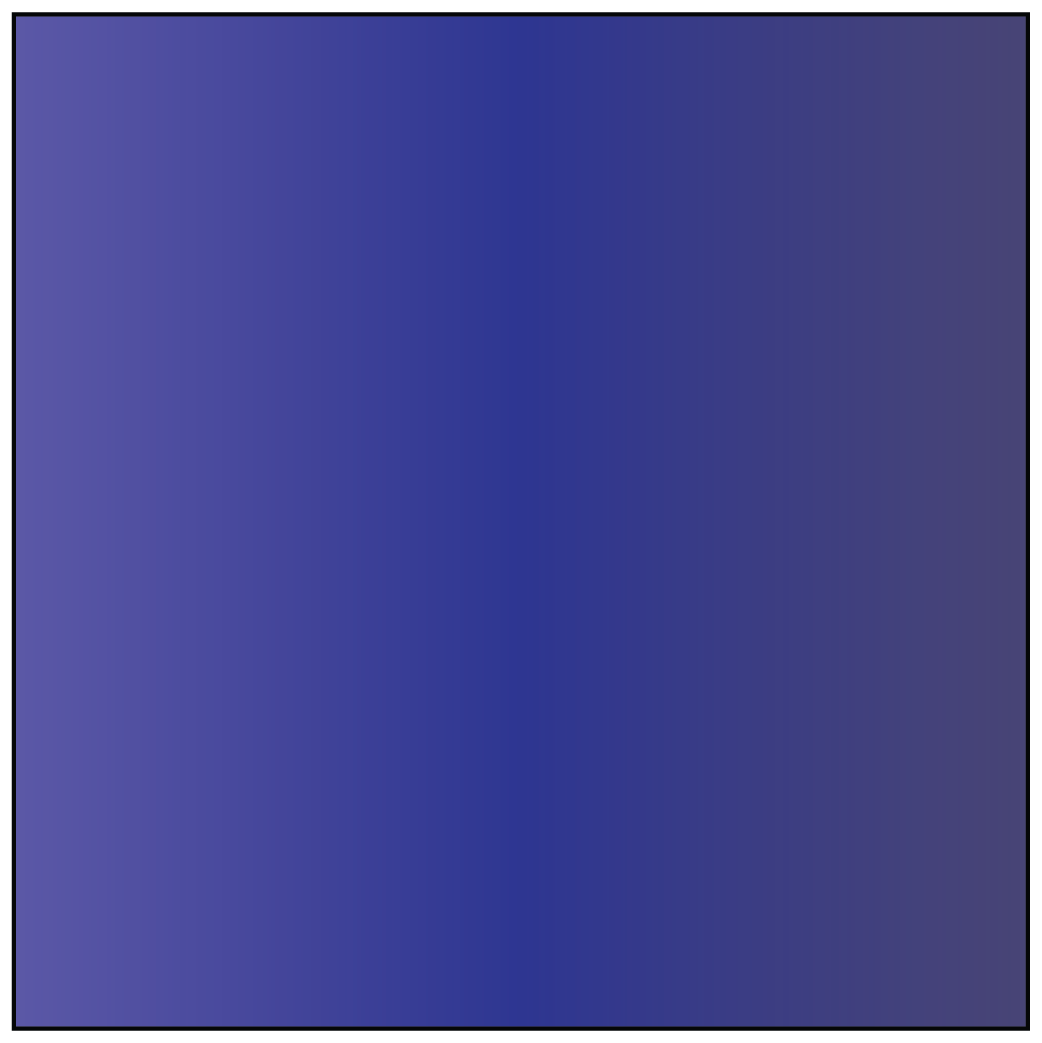Tetragon

5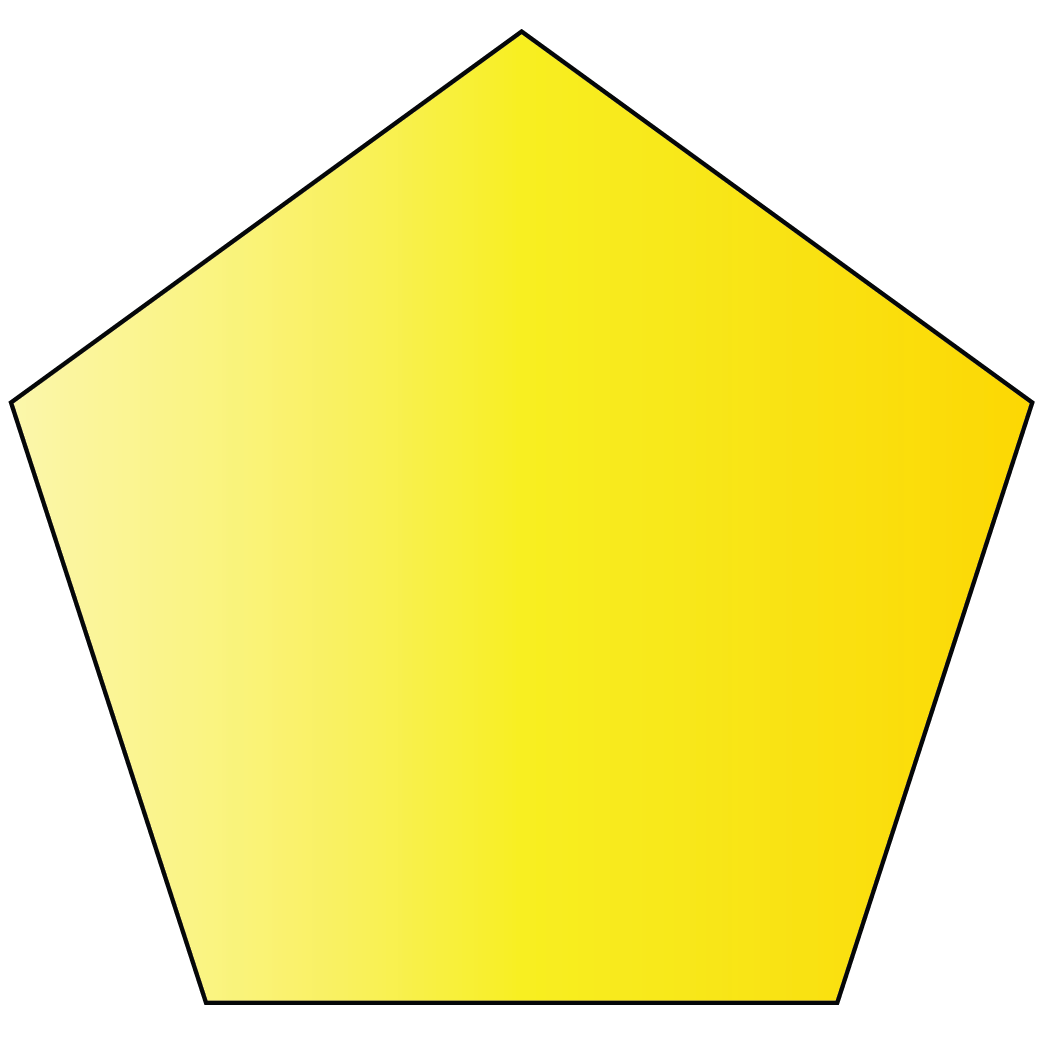Pentagon

6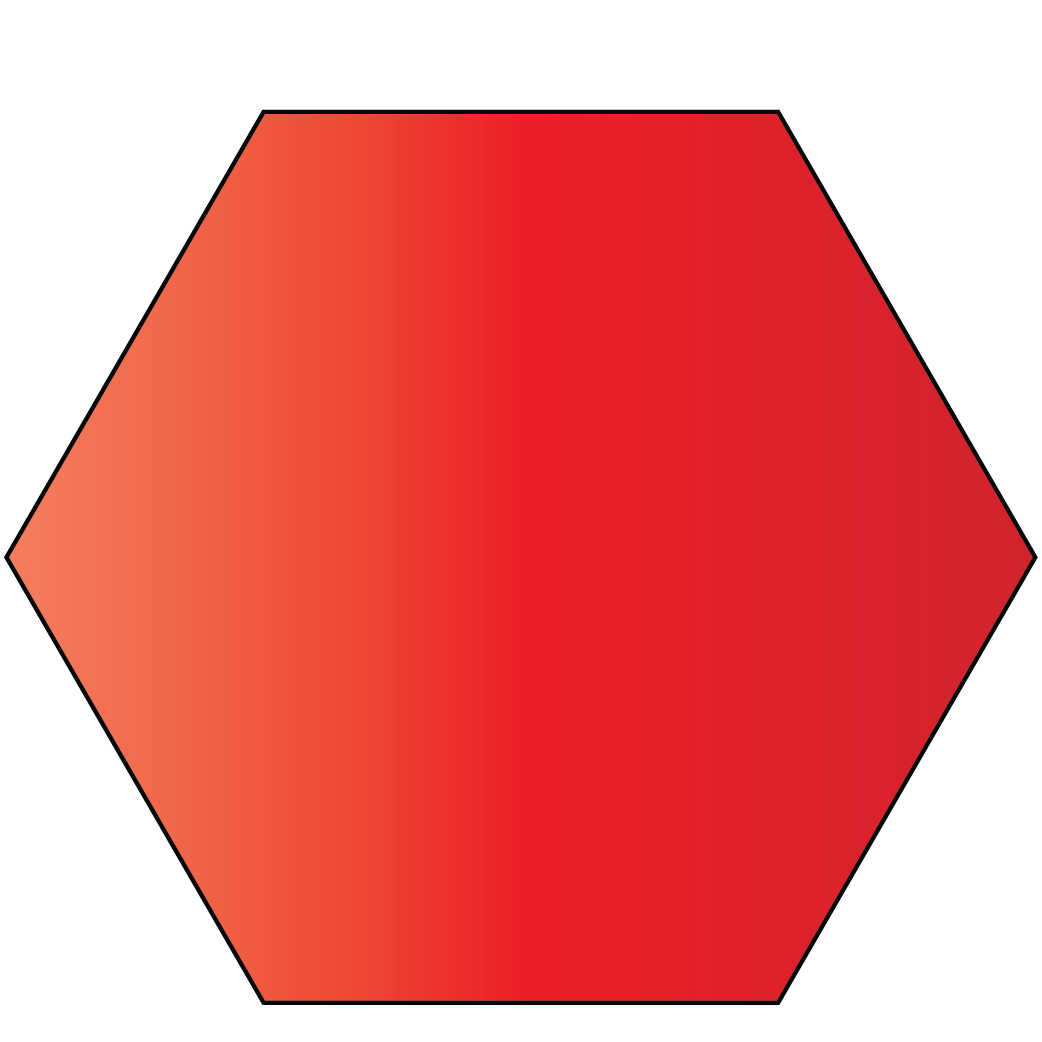Hexagon

7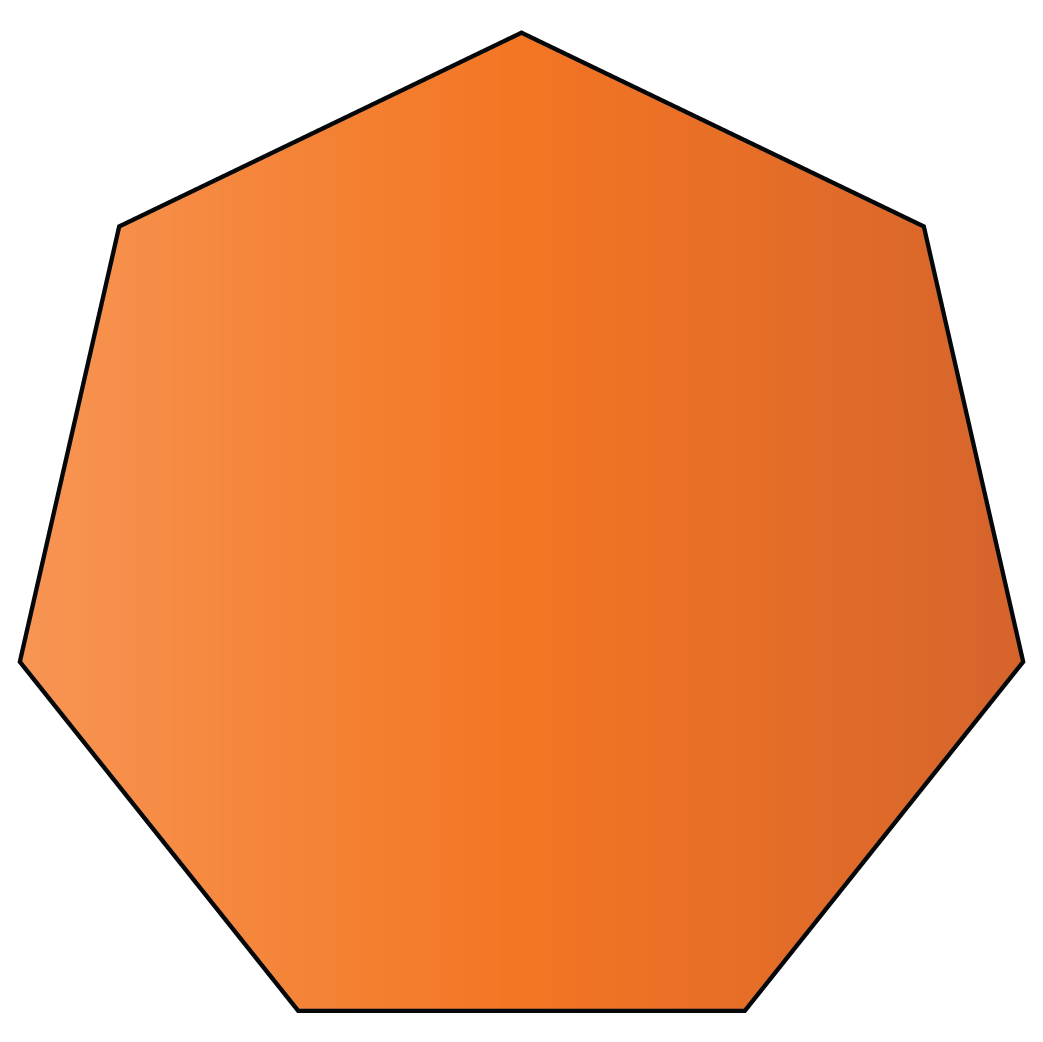Heptagon

8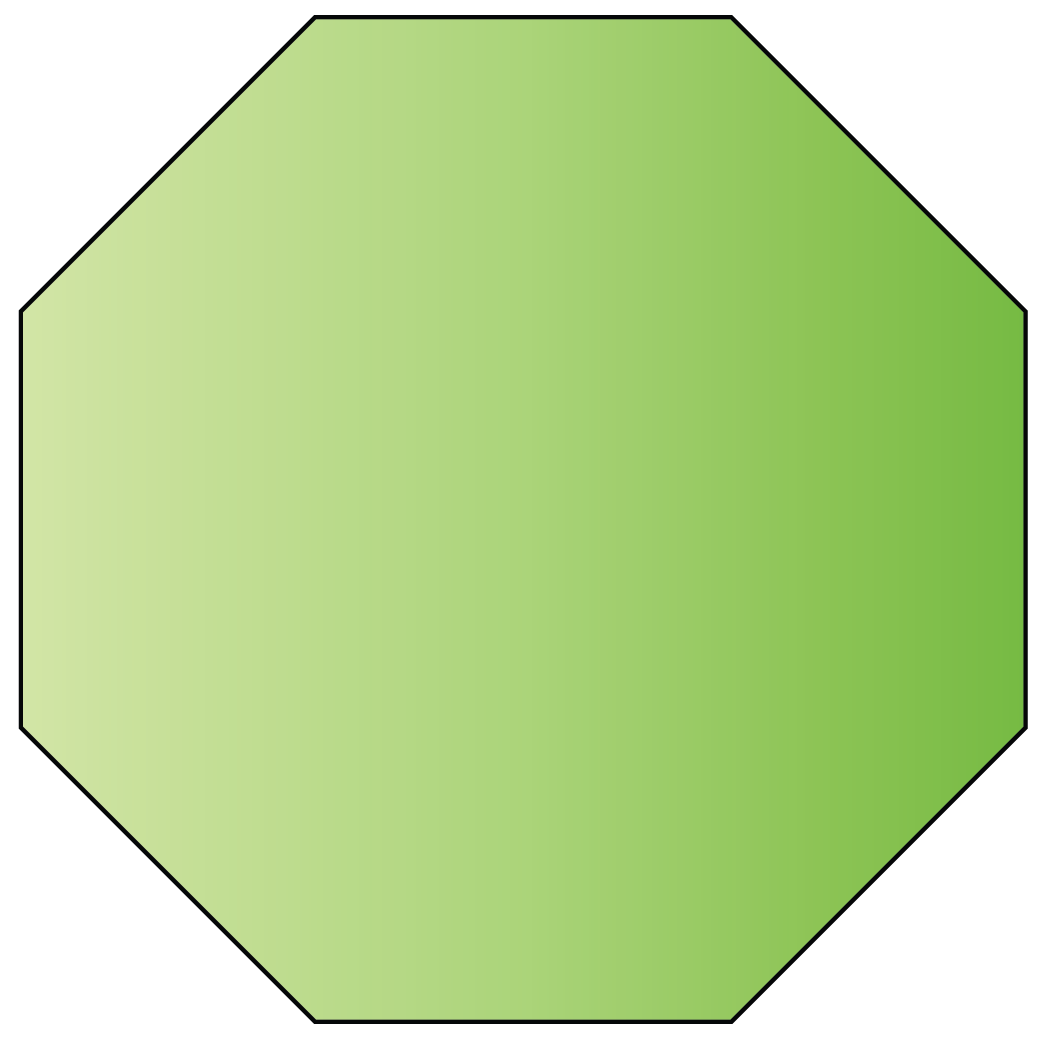Octagon

9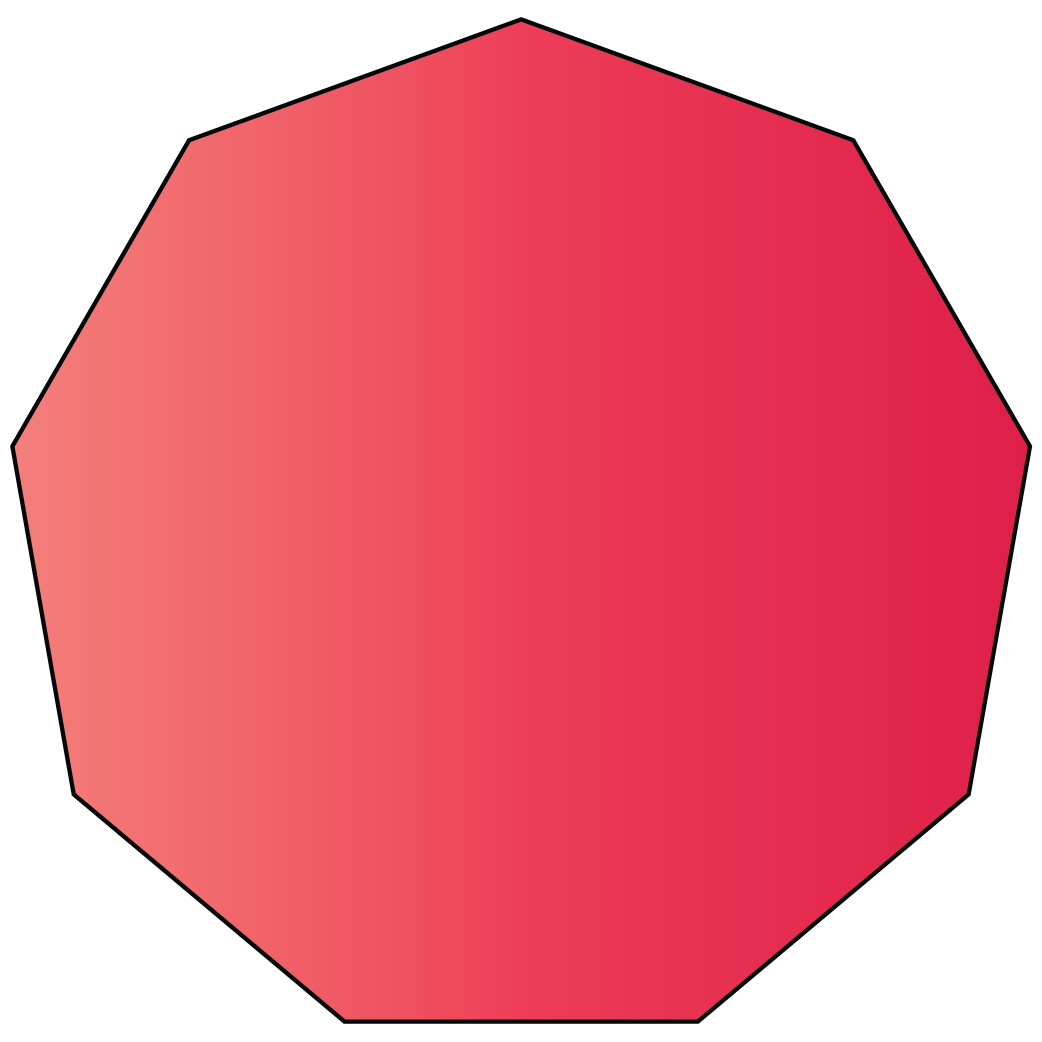Nonagon

10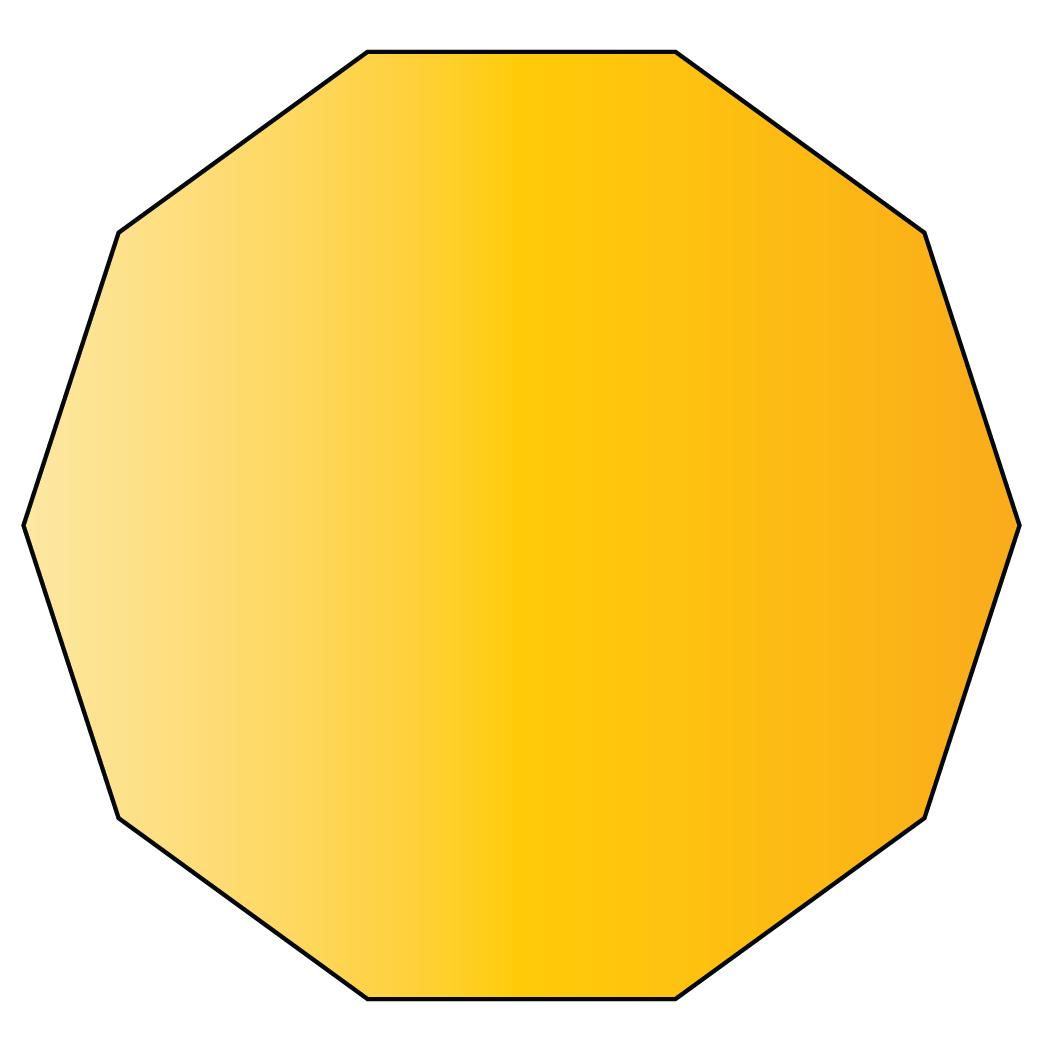Decagon

11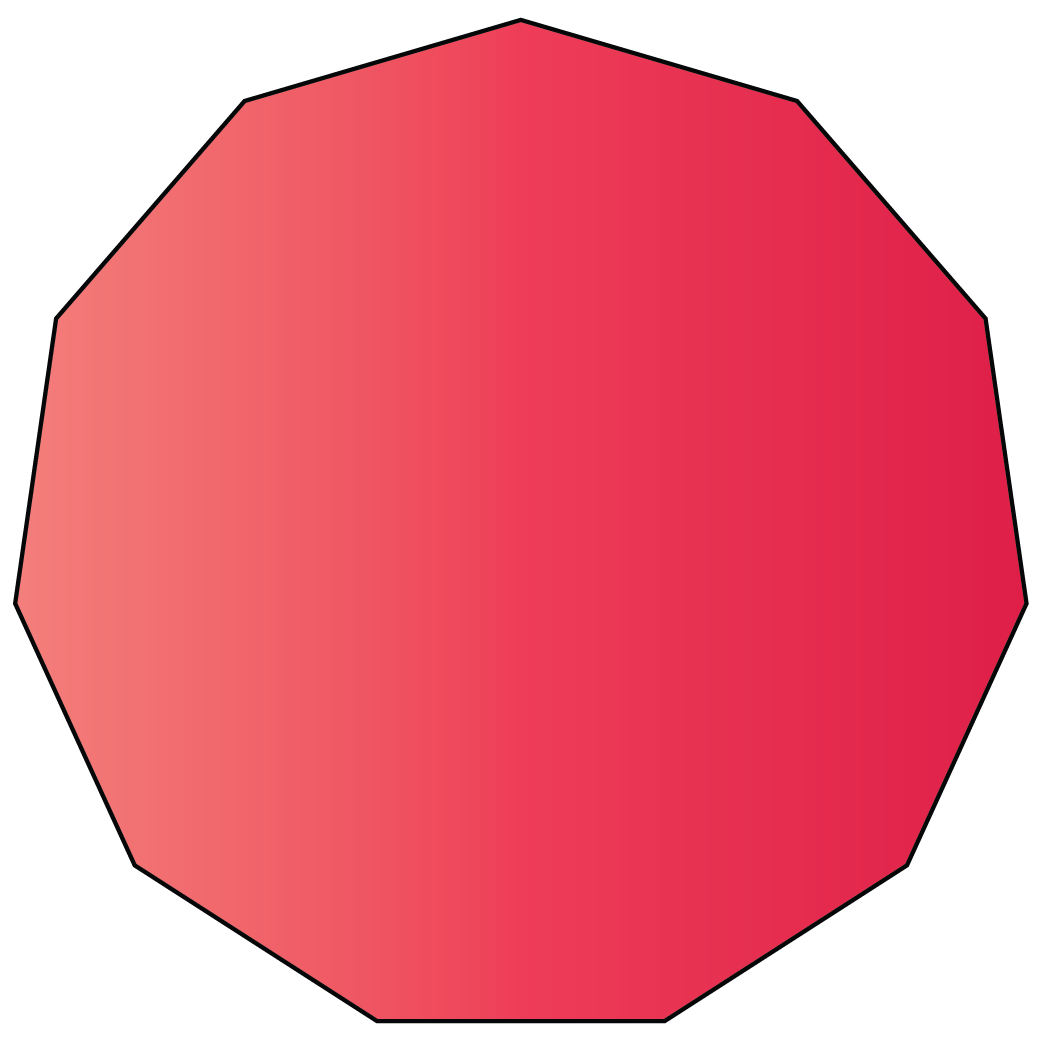Hendecagon

12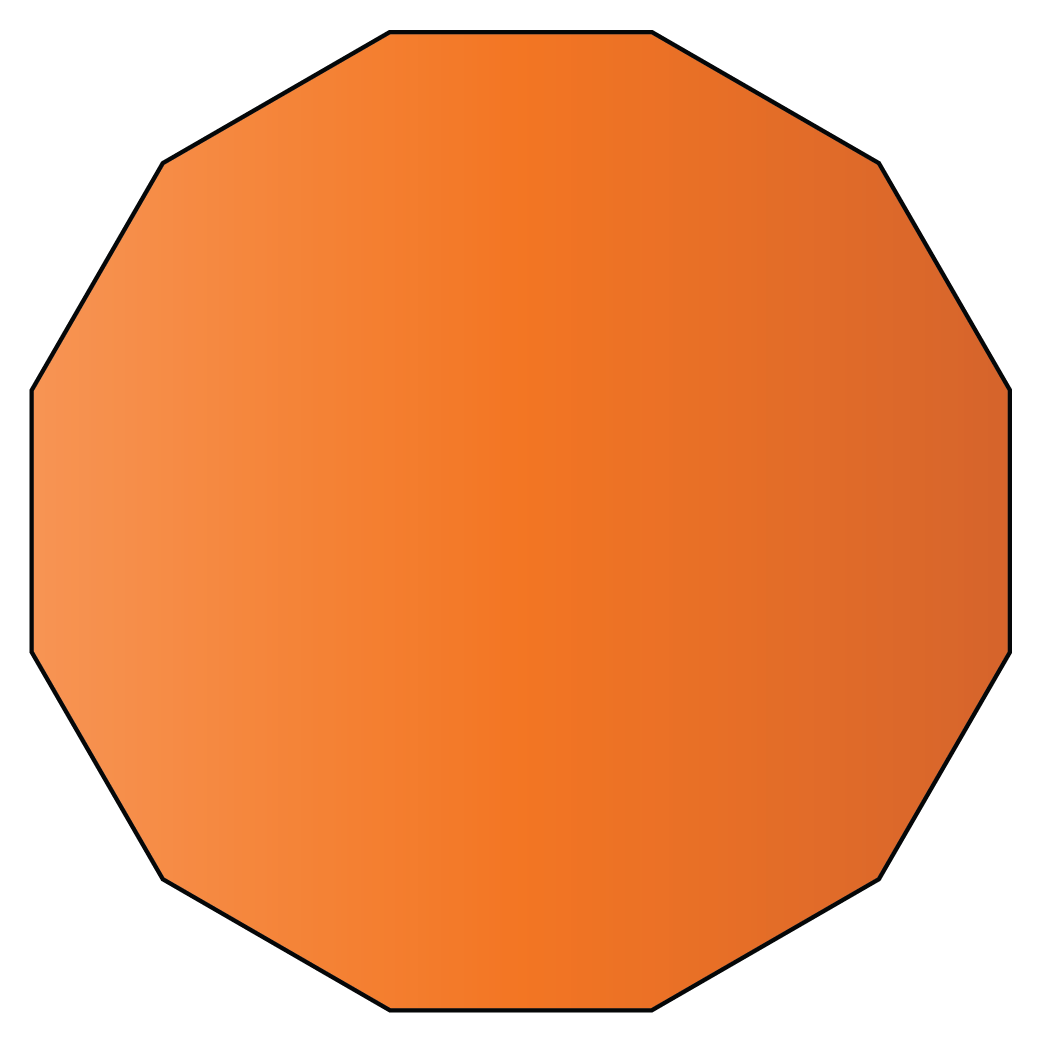Dodecagon

13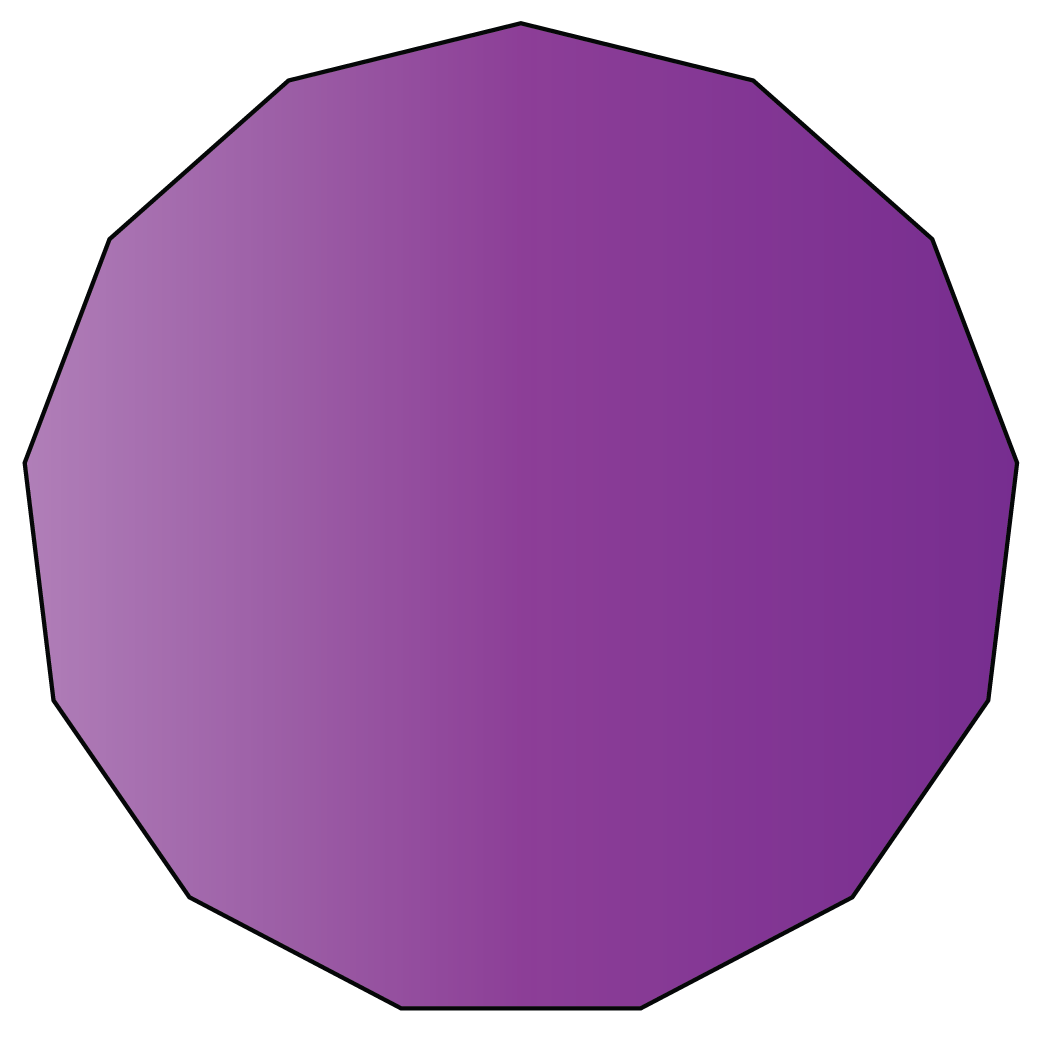Tridecagon

14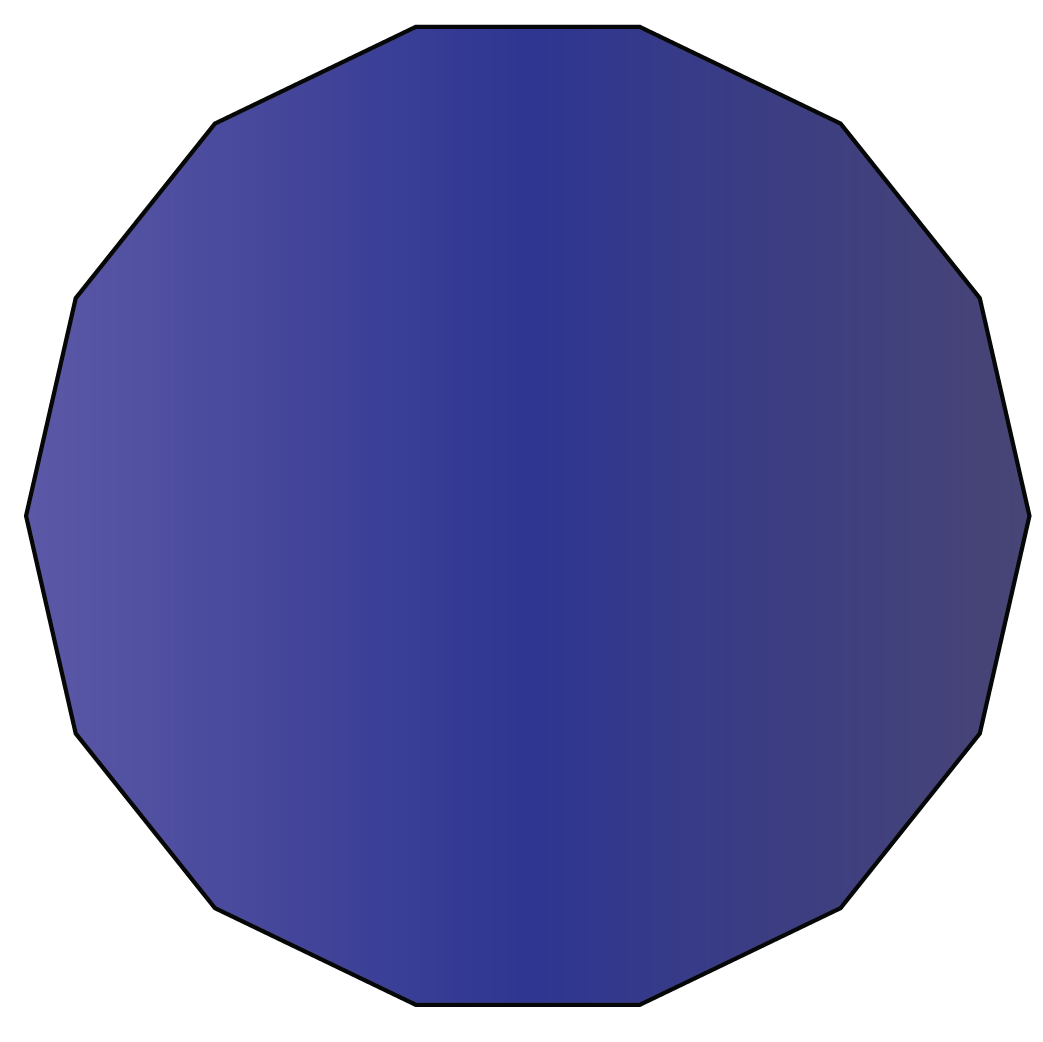15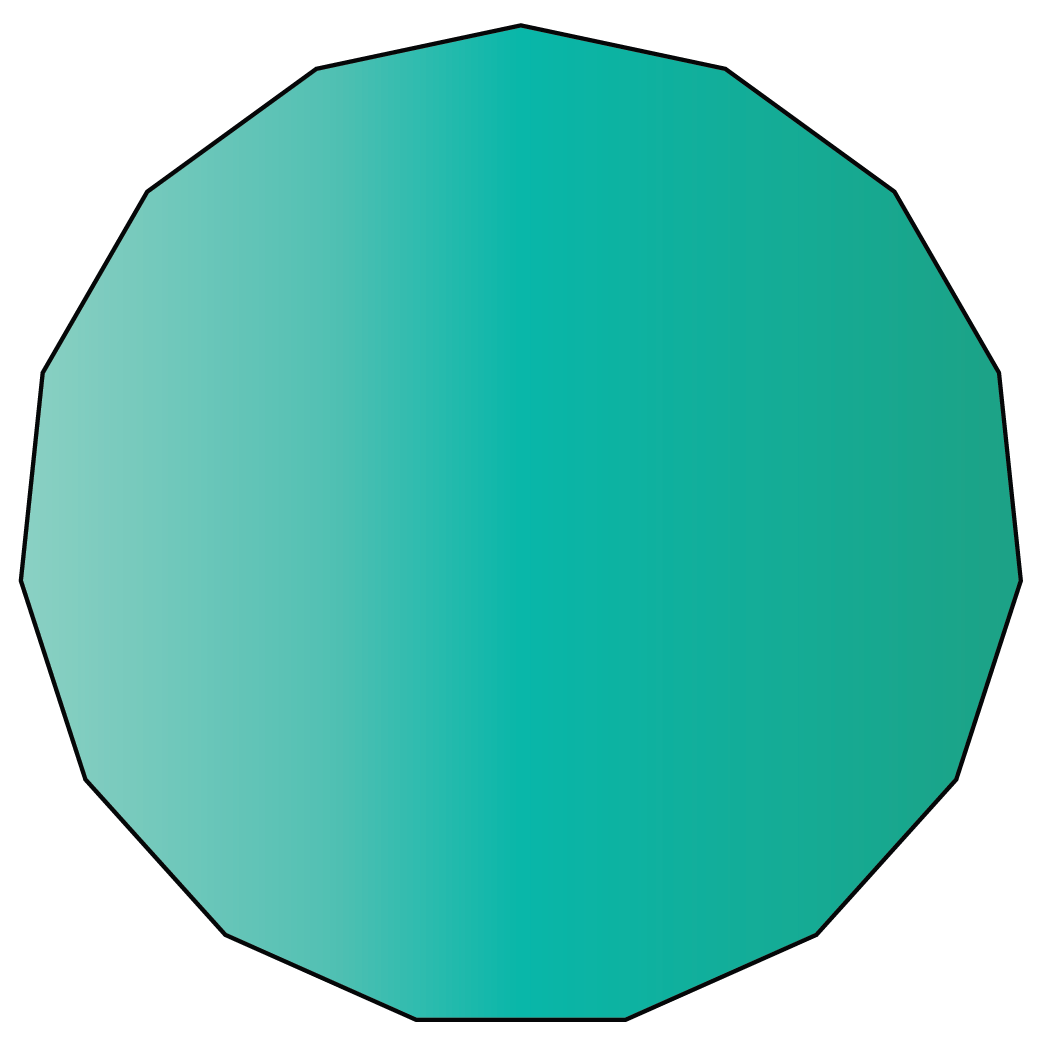16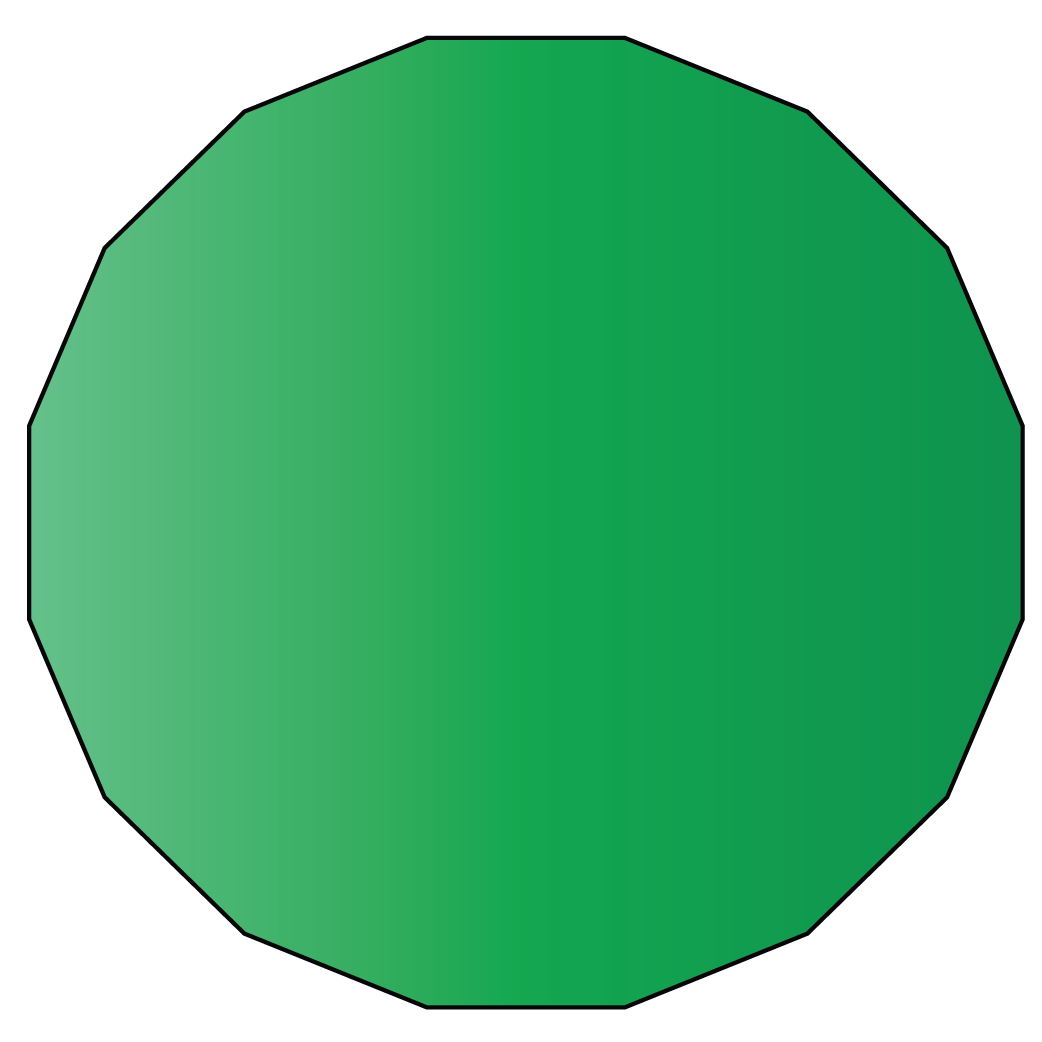17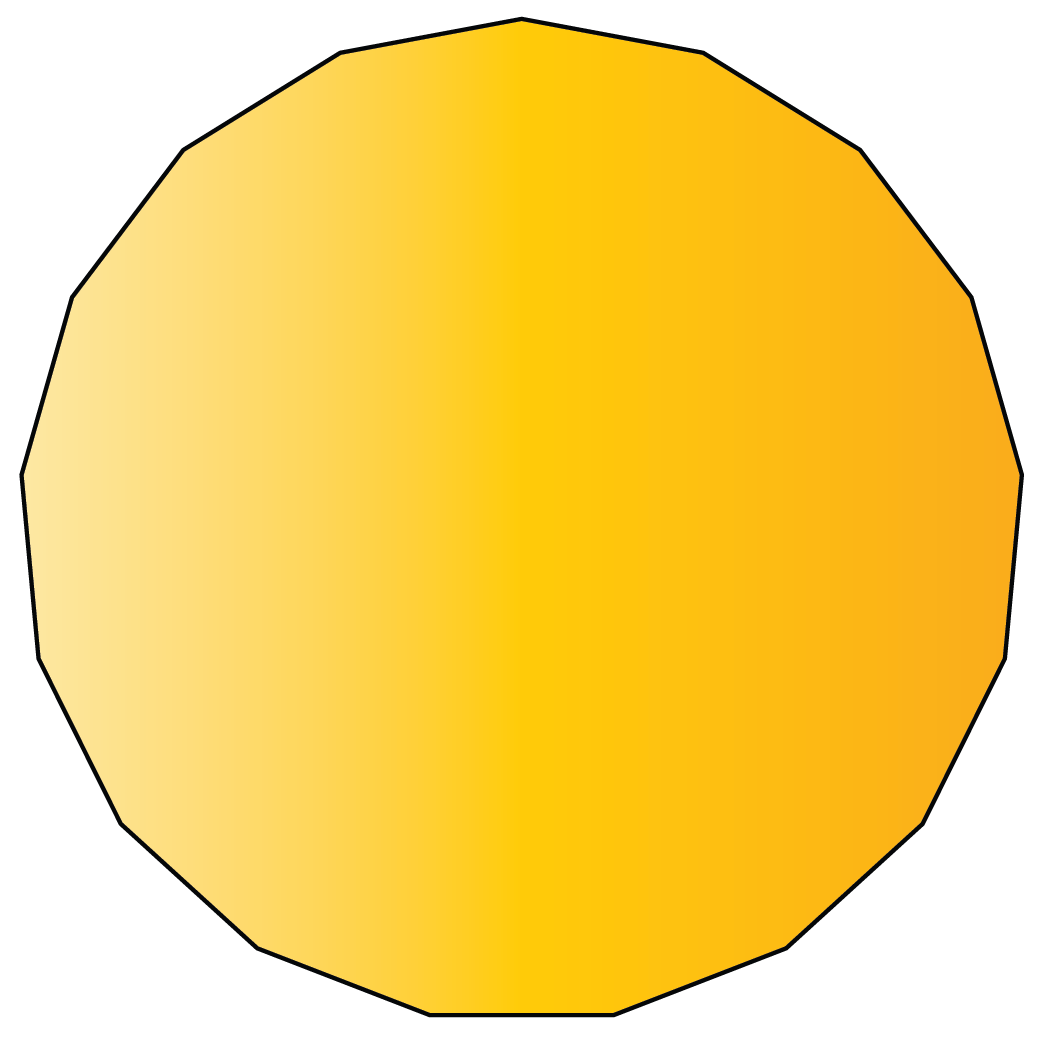18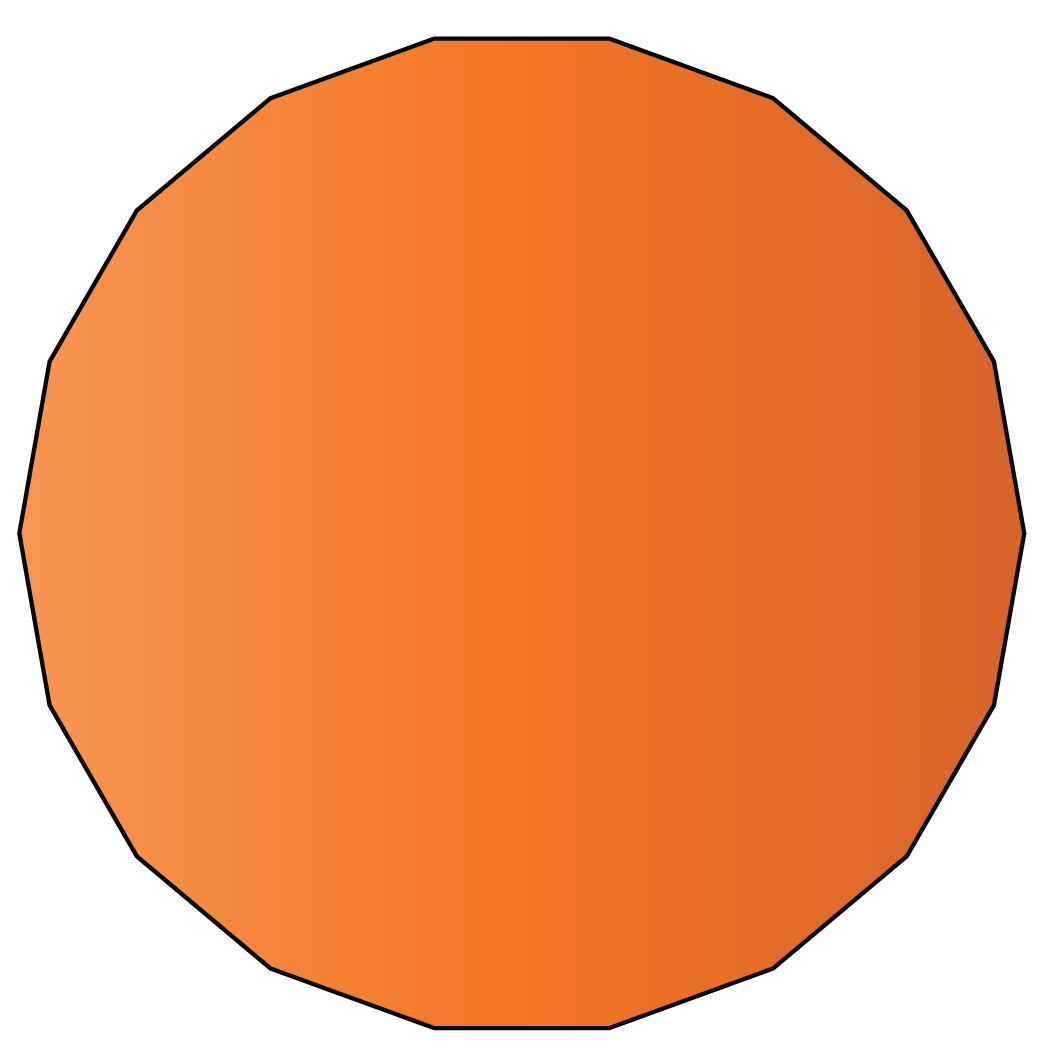19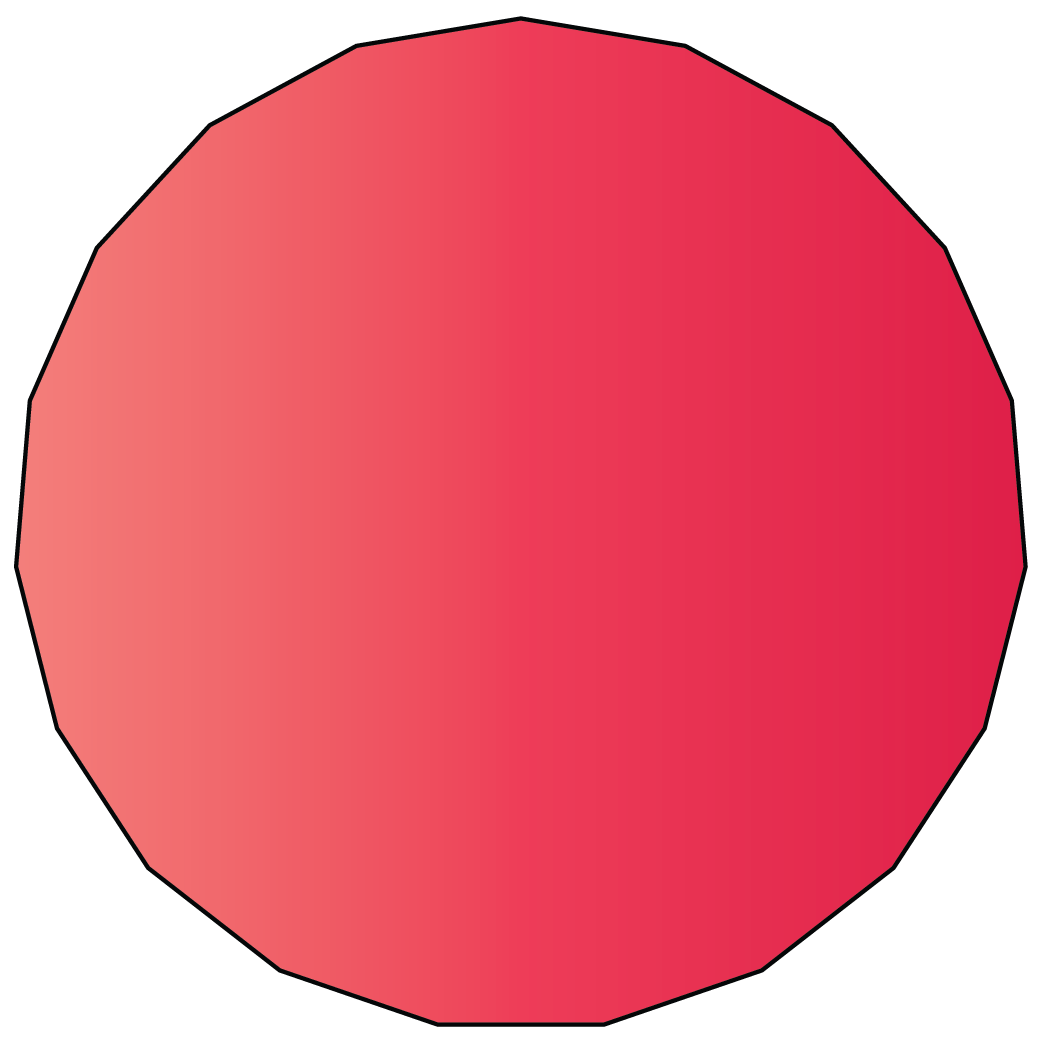20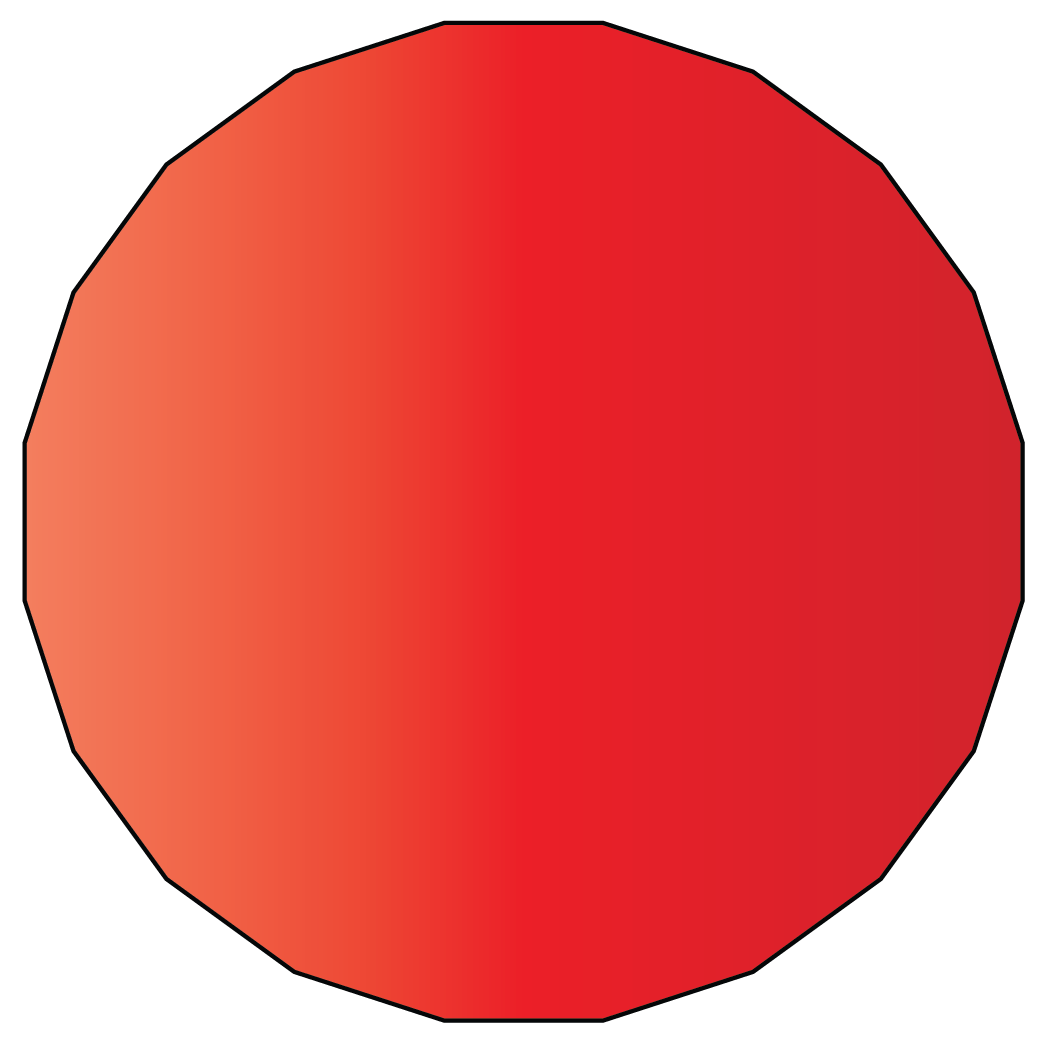Icosagon

## What Are the Properties of Shapes?

The properties of different shapes are as follows:

2D shape Property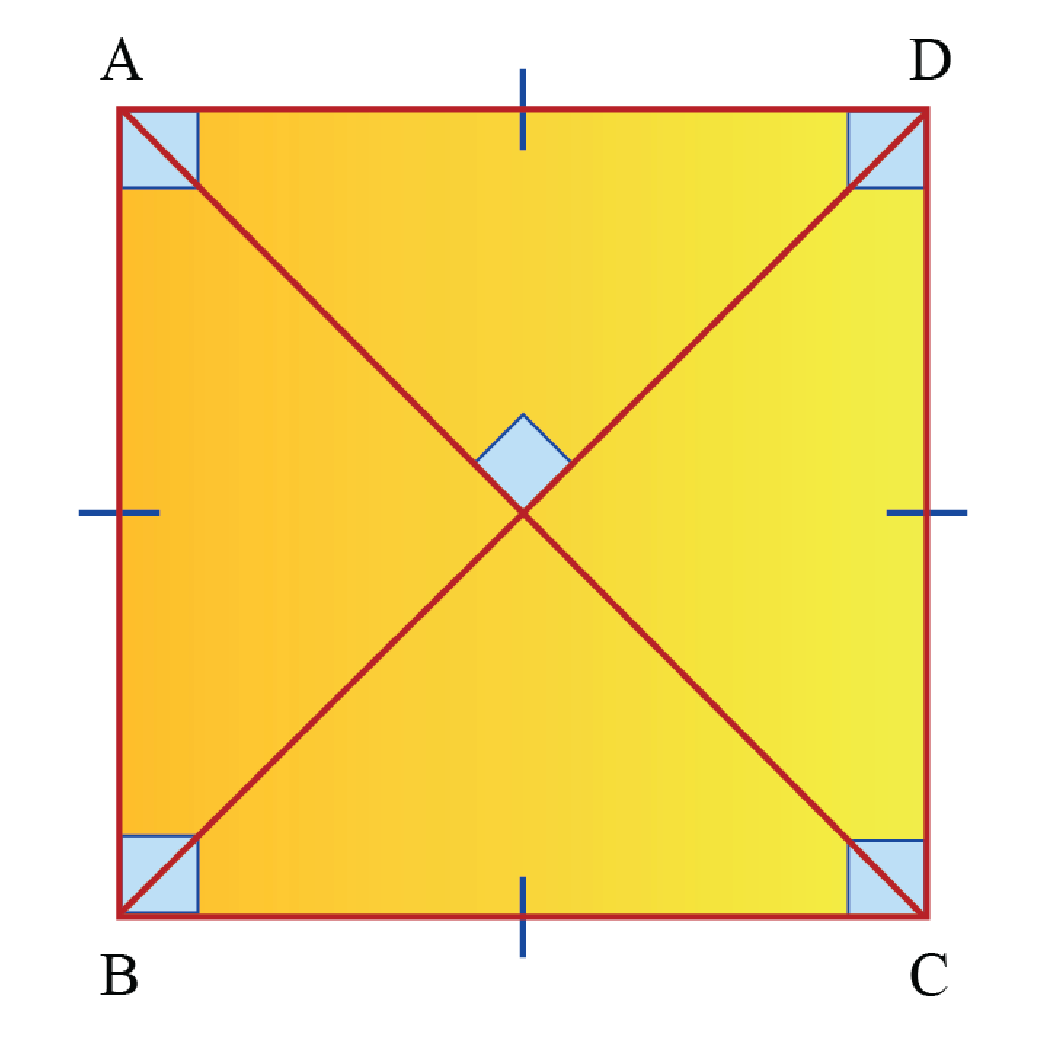Square

A square is a quadrilateral with four equal sides and four right angles.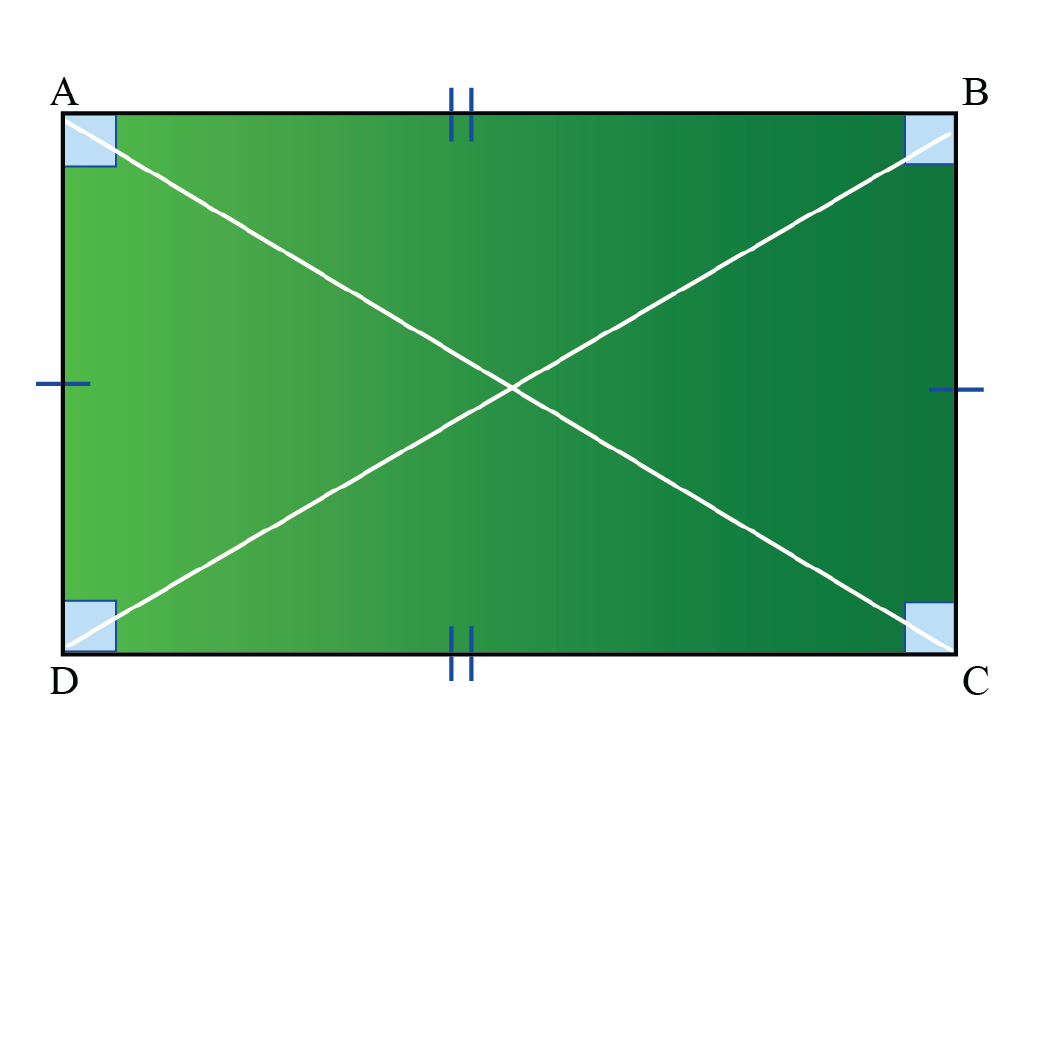Rectangle

A rectangle is a quadrilateral with two pairs of equal and parallel opposite sides and four right angles.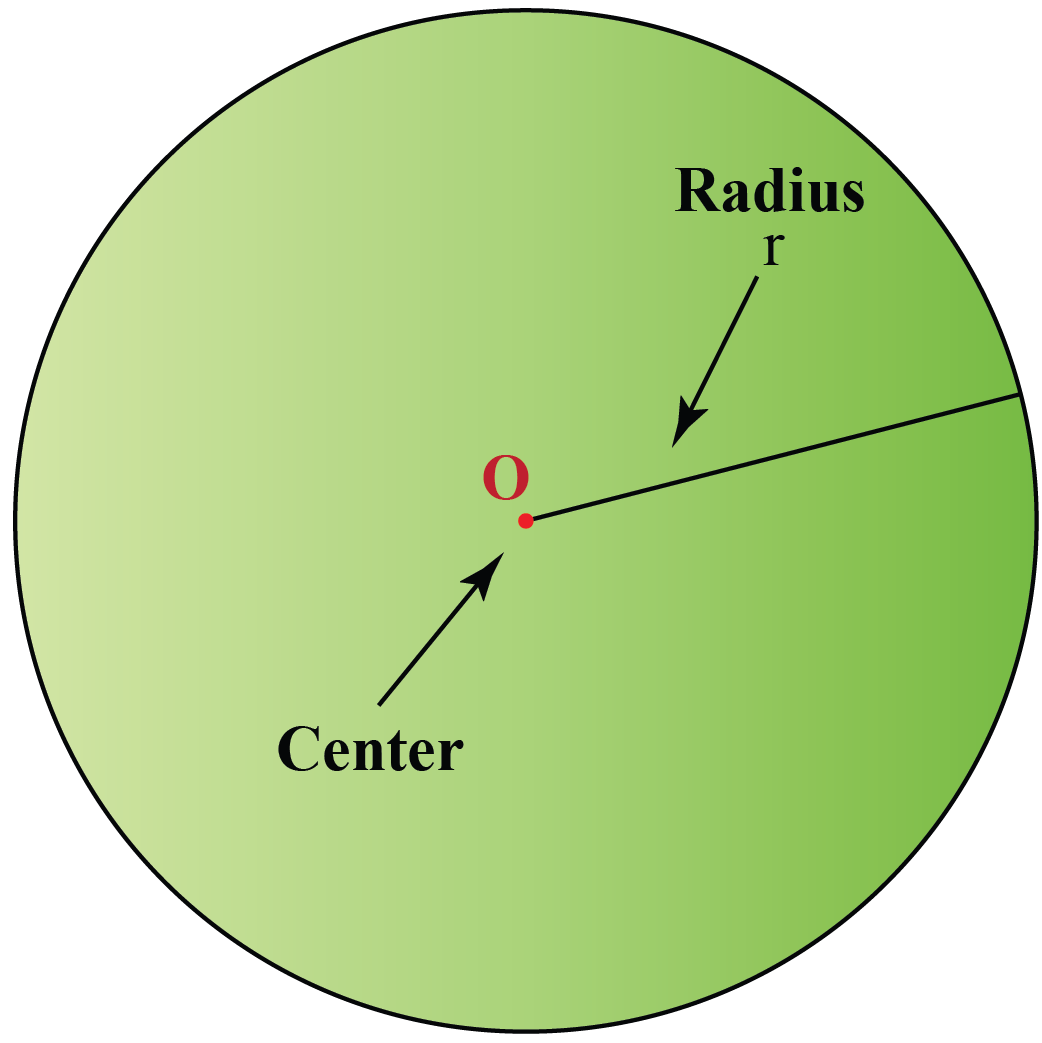Circle

circle is formed by the set of points which are at a constant or fixed distance (radius) from a fixed point (center) in the plane.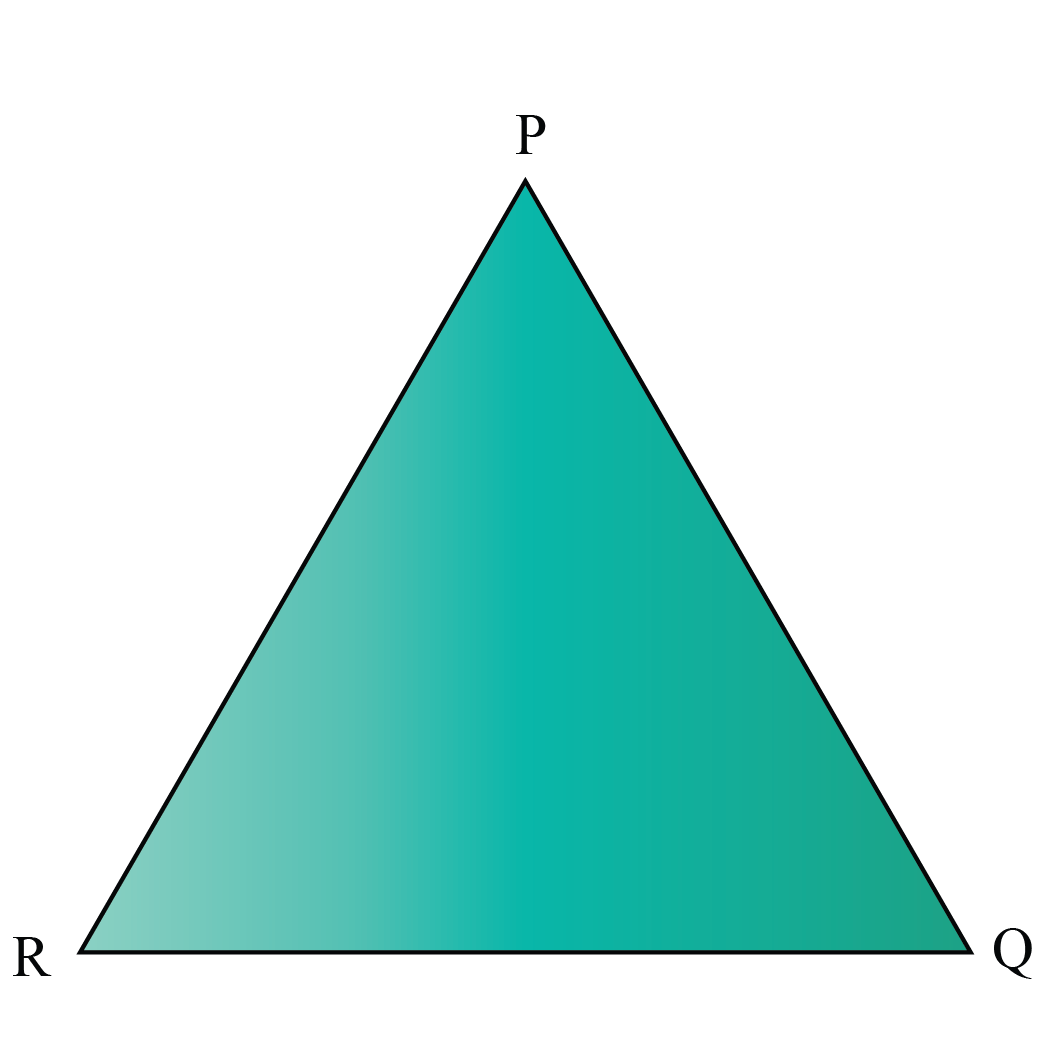Triangle

A triangle is a closed figure or shape with 3 sides, 3 angles, and 3 vertices.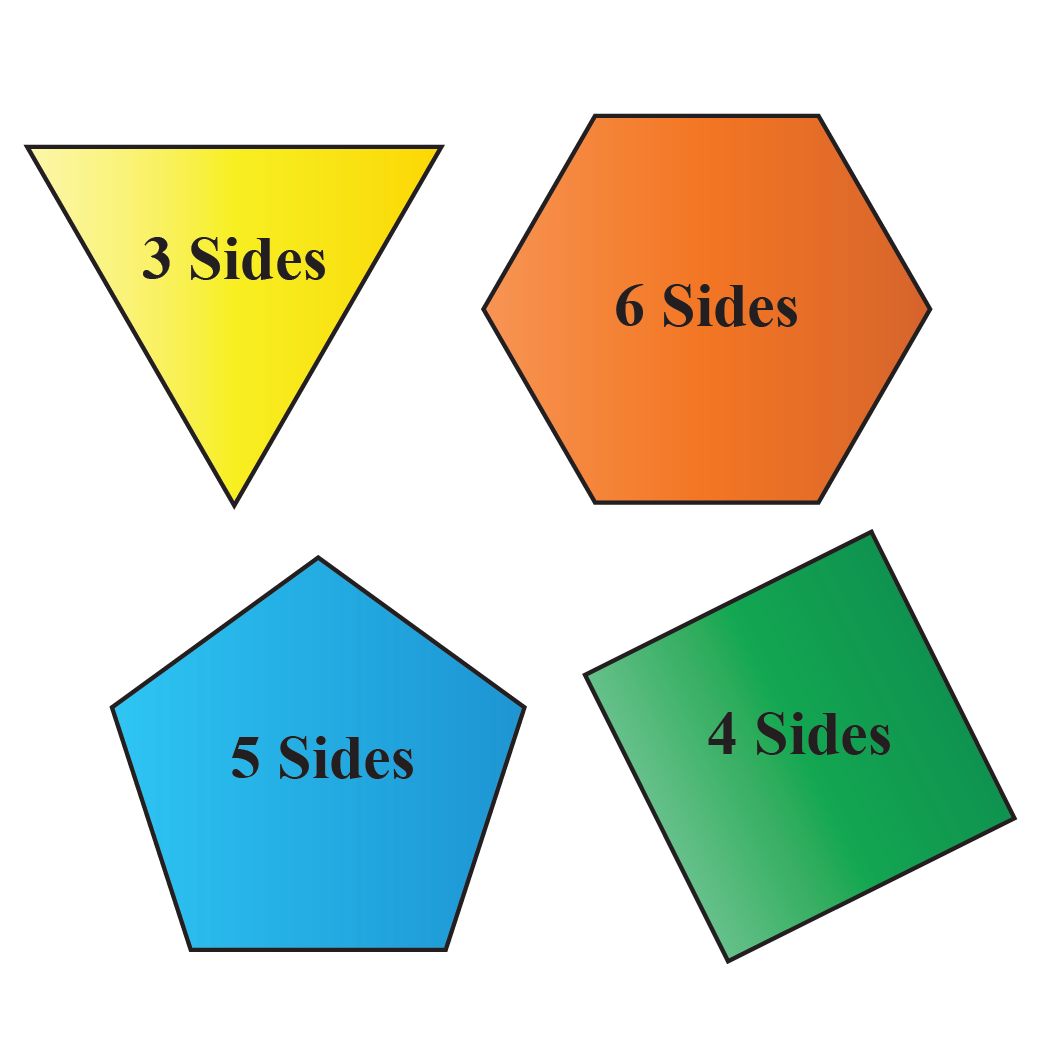Polygon

A polygon is a closed two-dimensional figure with three or more straight lines.Oval

Oval has no straight line, or corner, or side to it. It is a closed shape made of curved lines.

Here are a few activities for you to practice.Challenging Questions
1. Is a circle a polygon?
2. Is the given shape a quadrilateral?
3.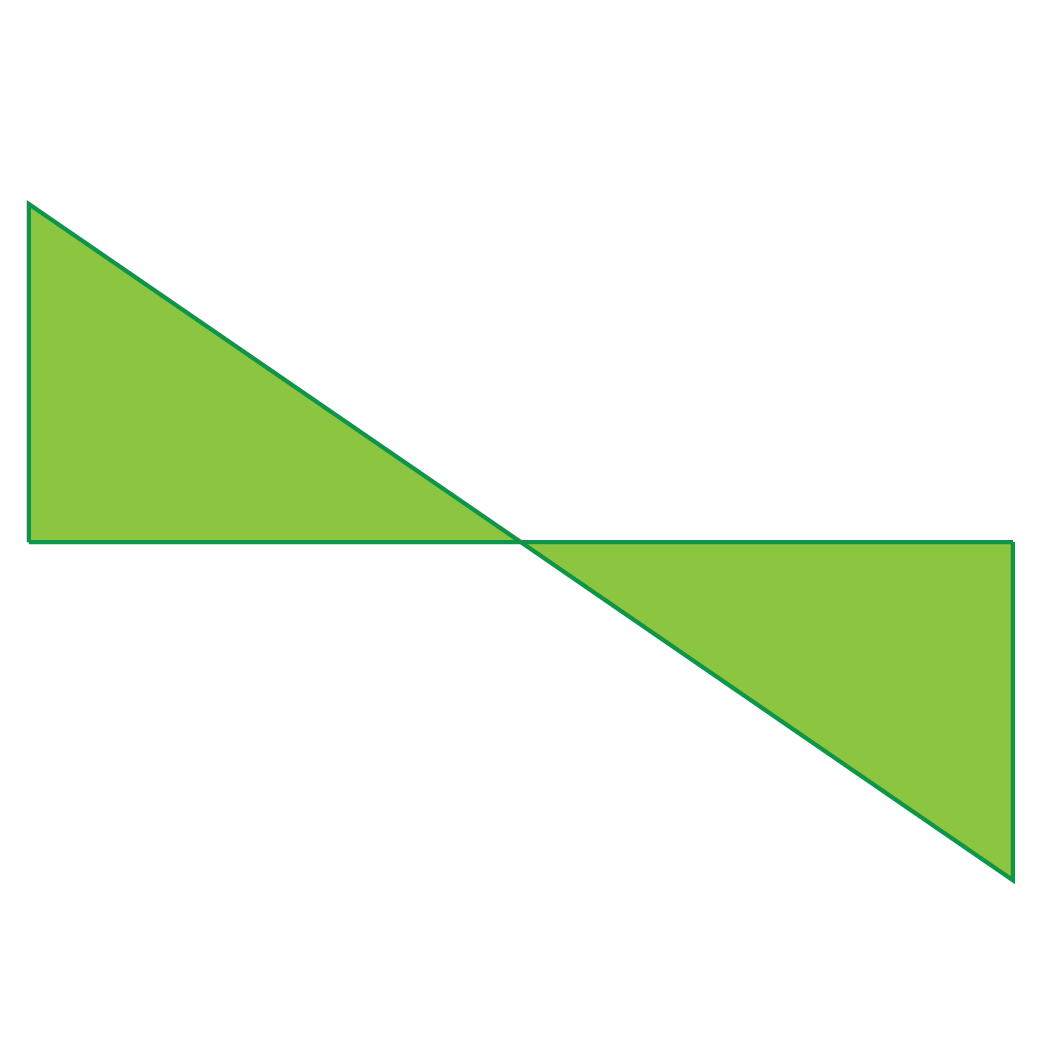## Let's Summarize

The mini-lesson targeted the fascinating concept of plane shapes. The math journey around plane shapes started with what a student already knew and went on to creatively crafting a fresh concept in the young minds. Done in a way that not only it is relatable and easy to grasp, but also will stay with them forever.

At Cuemath, our team of math experts is dedicated to making learning fun for our favorite readers, the students!

Through an interactive and engaging learning-teaching-learning approach, the teachers explore all angles of a topic.

Be it worksheets, online classes, doubt sessions, or any other form of relation, it’s the logical thinking and smart learning approach that we, at Cuemath, believe in.

## FAQs on Plane Shapes

### 1. Is a circle a polygon?

A polygon is a closed shape formed by straight lines.

A circle is a round shape formed by curved lines.

Therefore, a circle is not a polygon.

### 2. What is the difference between 2D and 3D shapes?

A 2D shape has two dimensions whereas a 3D shape has three dimensions - length, width, and height.

### 3. When depth is added to a 2D shape it becomes?

When depth is added to a 2D shape with length and width, it becomes a 3D shape.

### 4. How many triangles are there in a pentagon?

The number of triangles is 35 in a pentagon when all diagonals are formed.

More Important Topics
Numbers
Algebra
Geometry
Measurement
Money
Data
Trigonometry
Calculus
More Important Topics
Numbers
Algebra
Geometry
Measurement
Money
Data
Trigonometry
Calculus